# Tamil Nadu Board Question Papers for Class 12th Maths 2019 With Solutions In PDF

Tamil Nadu Class 12 Maths 2019 question paper with solutions are provided here in a downloadable pdf format and also in the text, so that the students can get an overview of the question paper design, the marks distribution and types of questions that could be asked in the exams. Along with the solutions, Maths question paper 2019 Class 12 is also added for reference. Students are able to access all the Tamil Nadu board previous year maths question papers here.

The solutions provided by BYJU’S will help in providing good practice for those students, who are preparing for the board exams. Solving 2019 Maths question papers and also the previous year papers for Class 12 is an ideal way to learn and revise for the exams. The solutions are prepared in easy language too.

Here, in this article please find the links to access the PDF formats of the Tamil Nadu Board Class 12 Maths Previous Year Question Paper 2019 with solutions or even just the question paper, as per preference.

TN Board Class 12th Maths Question Paper With Solutions 2019
QUESTION PAPER CODE 1312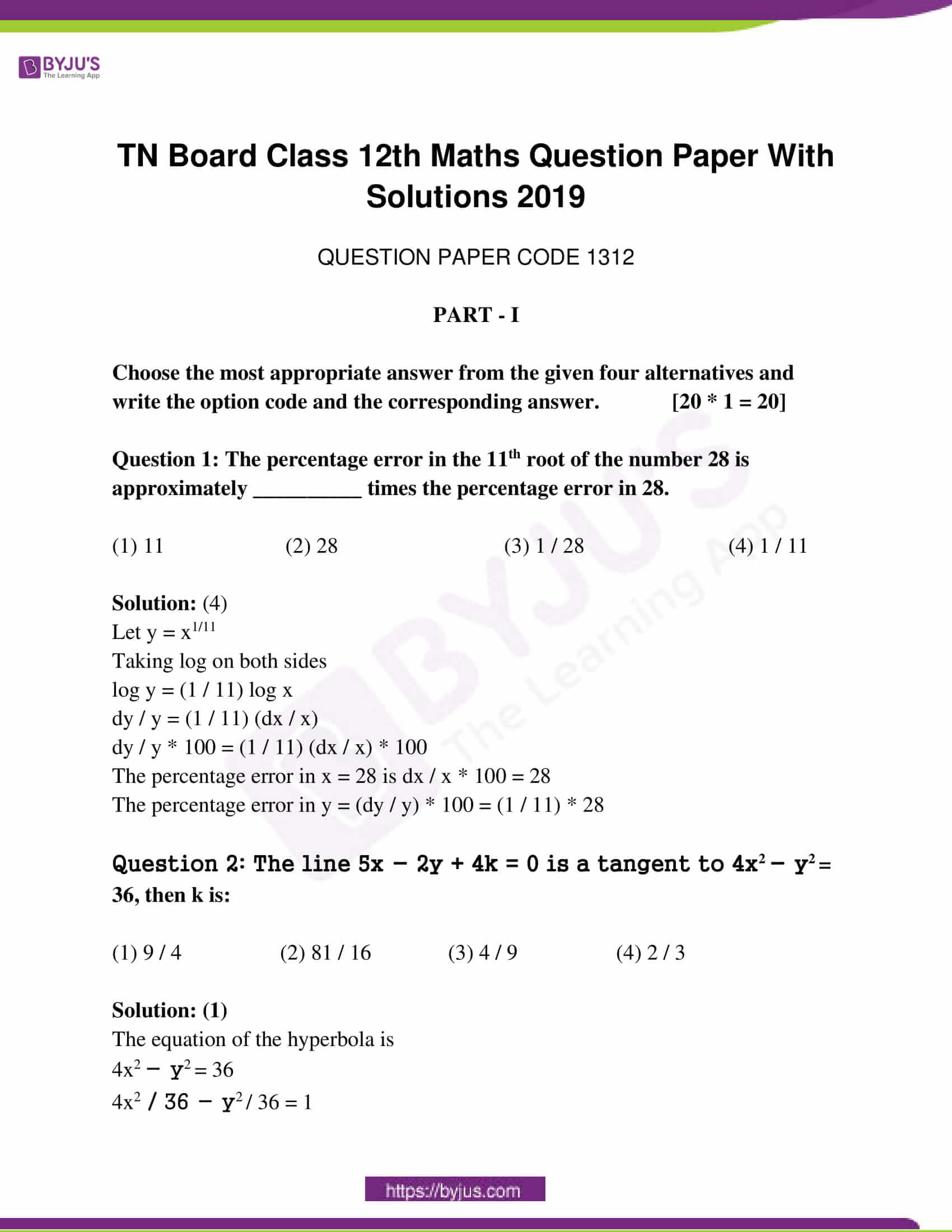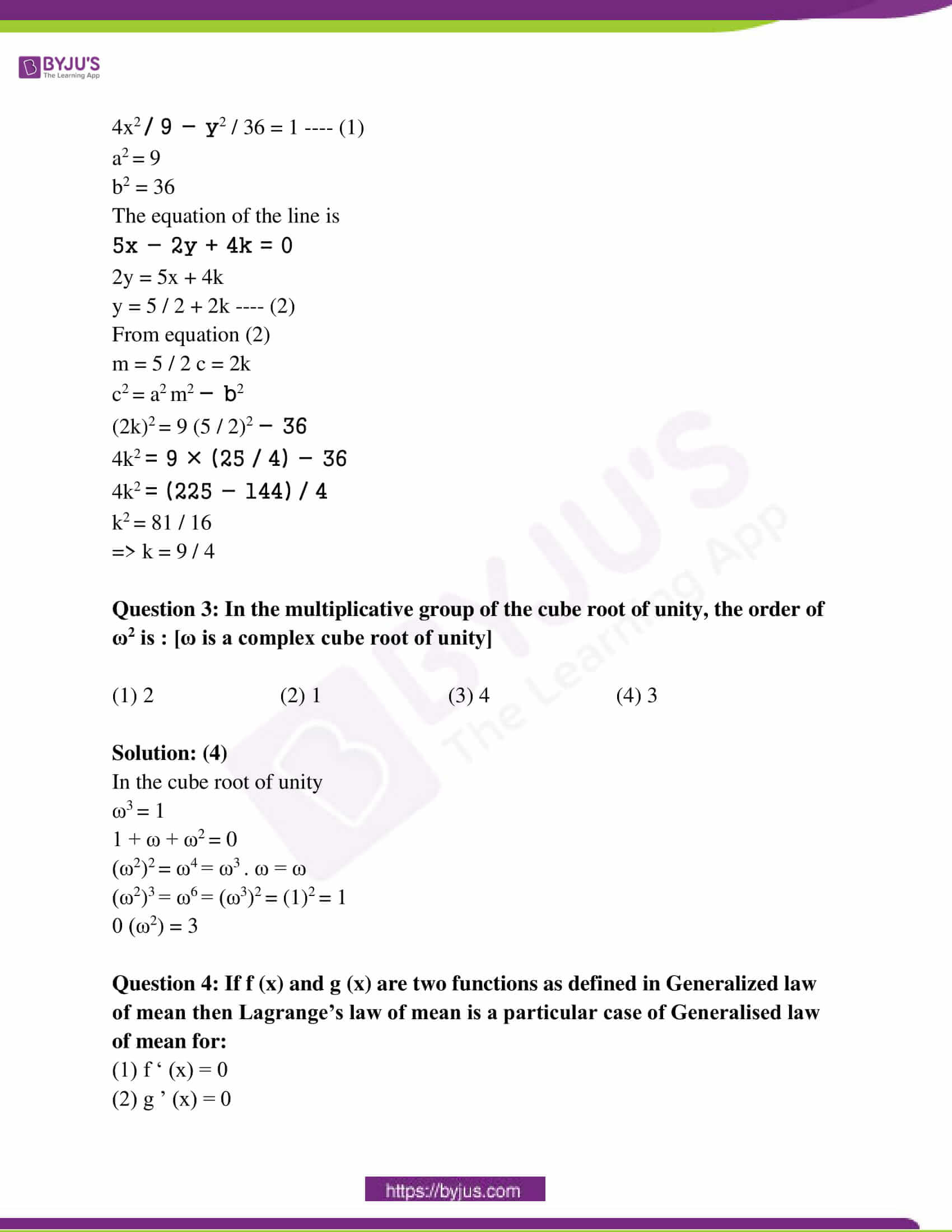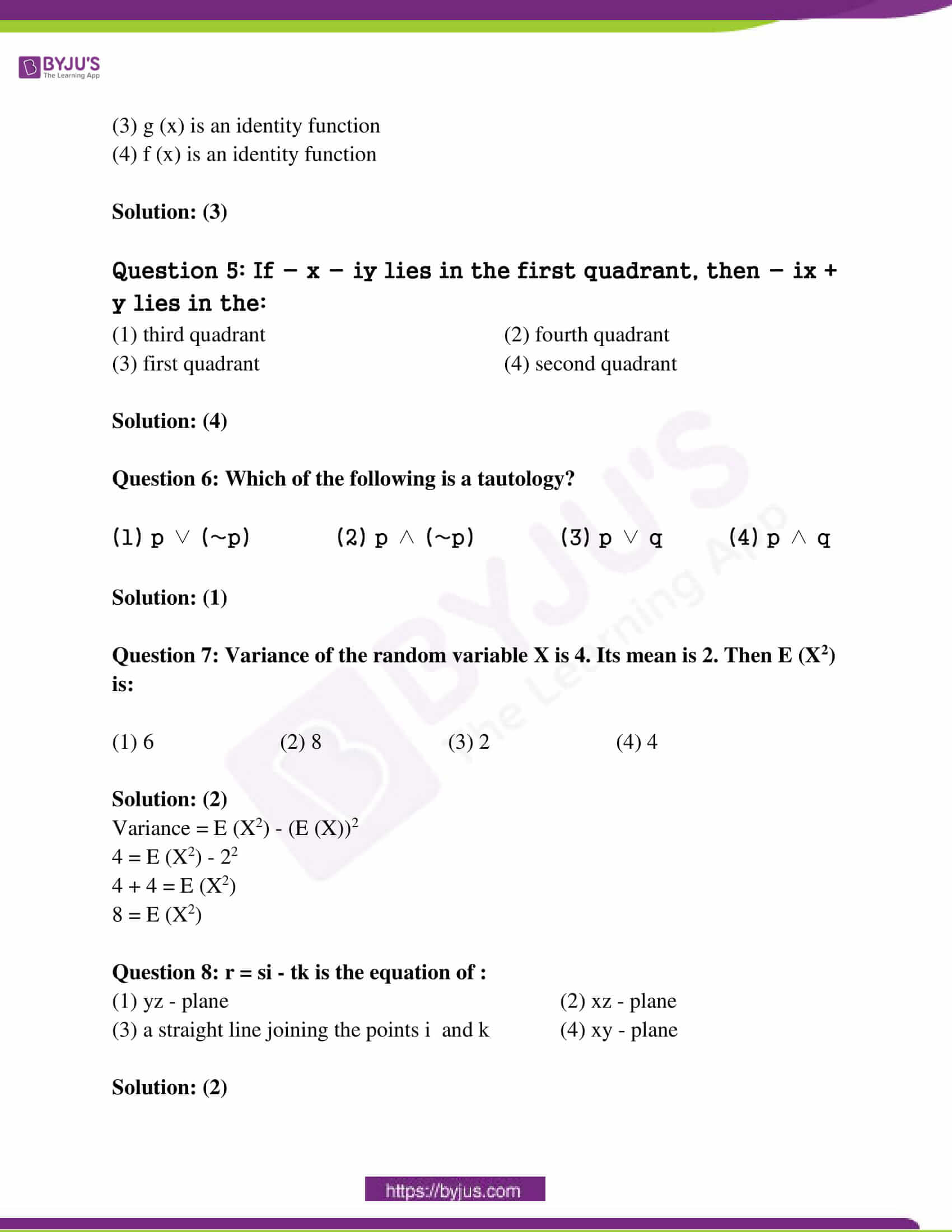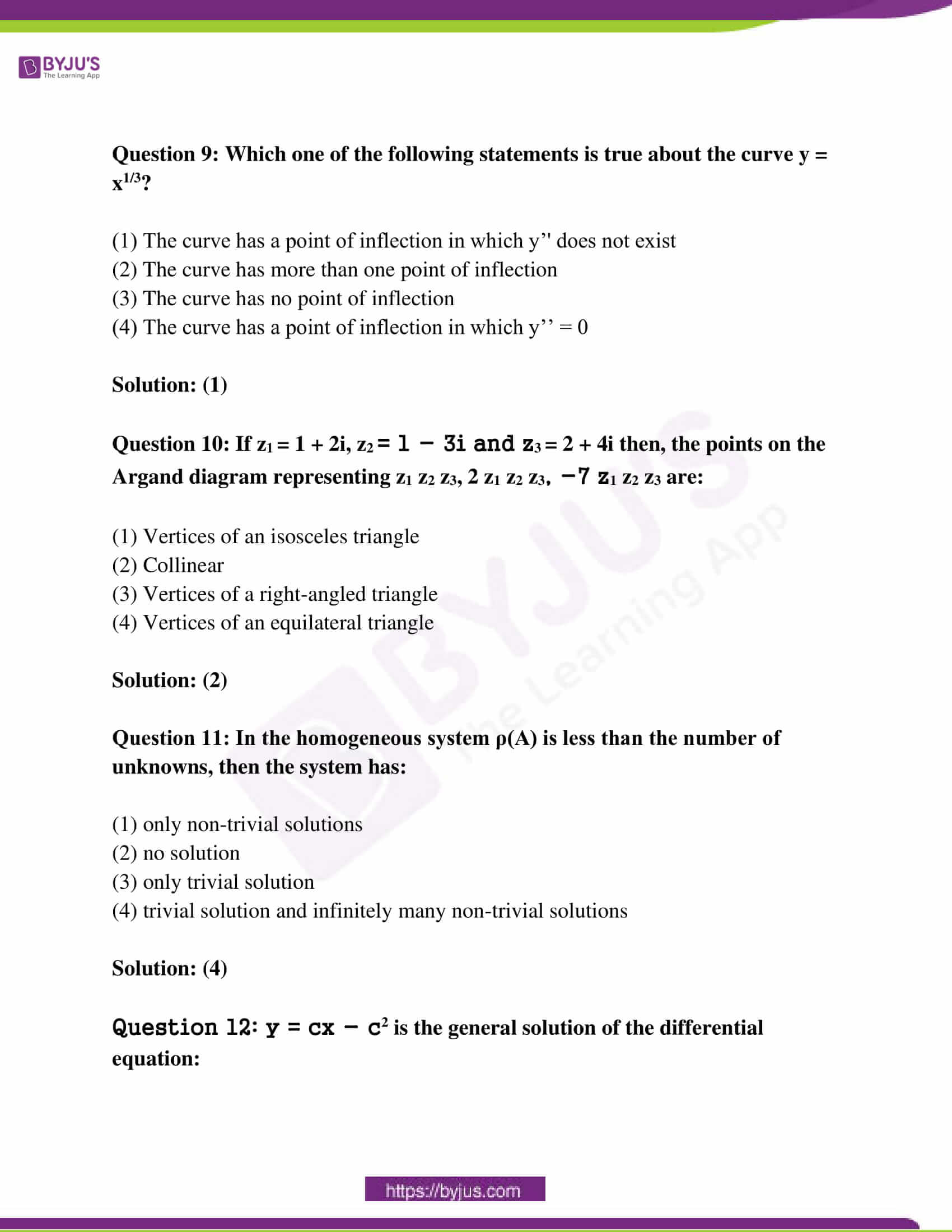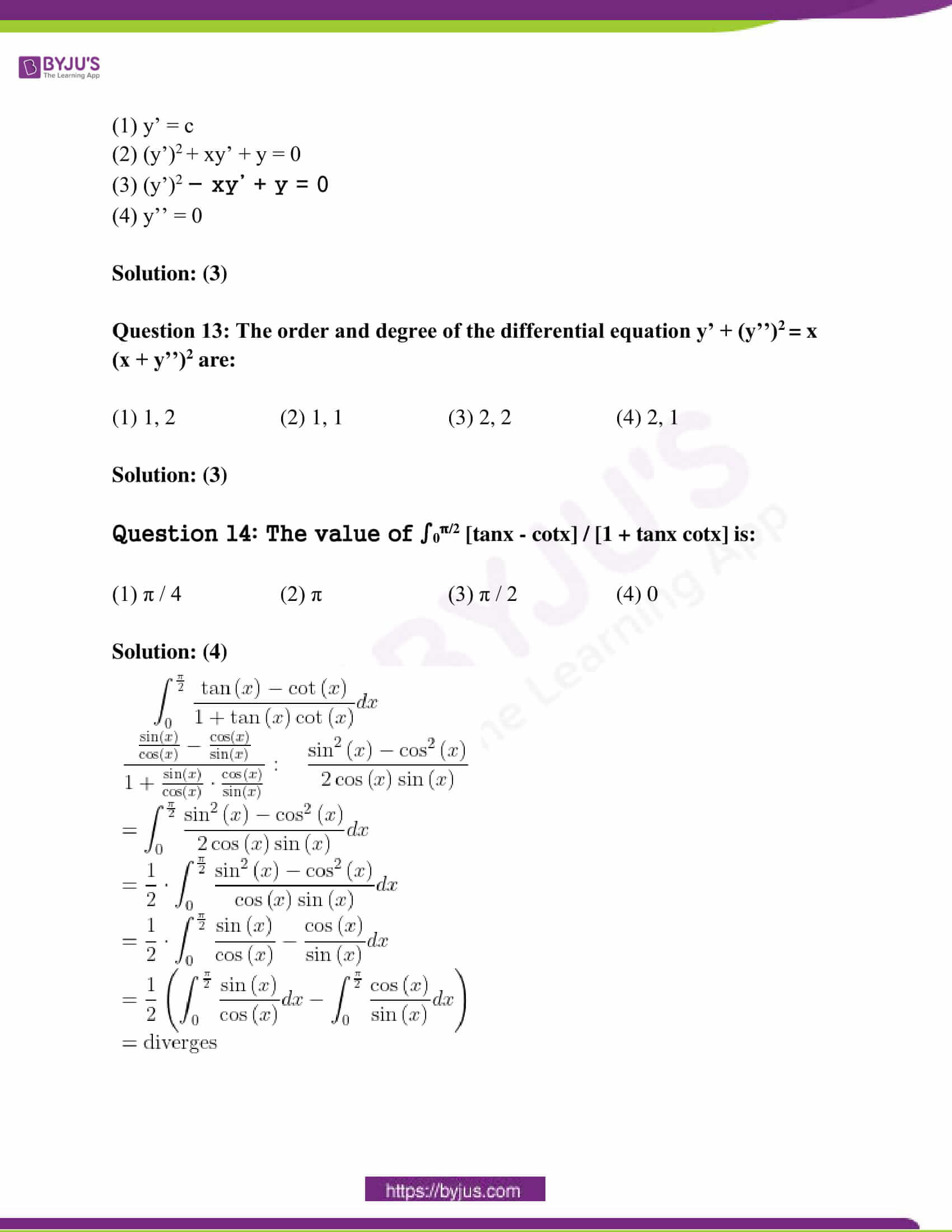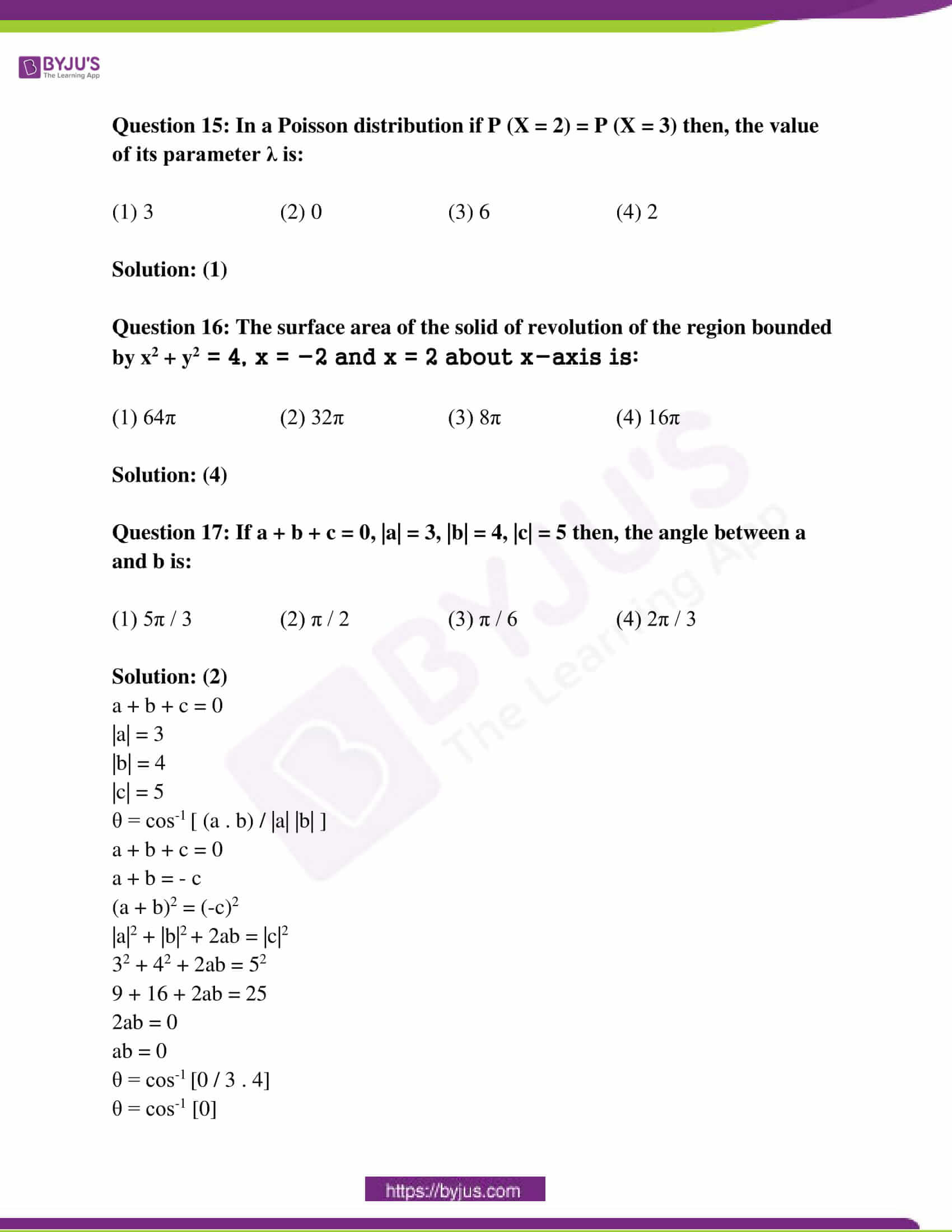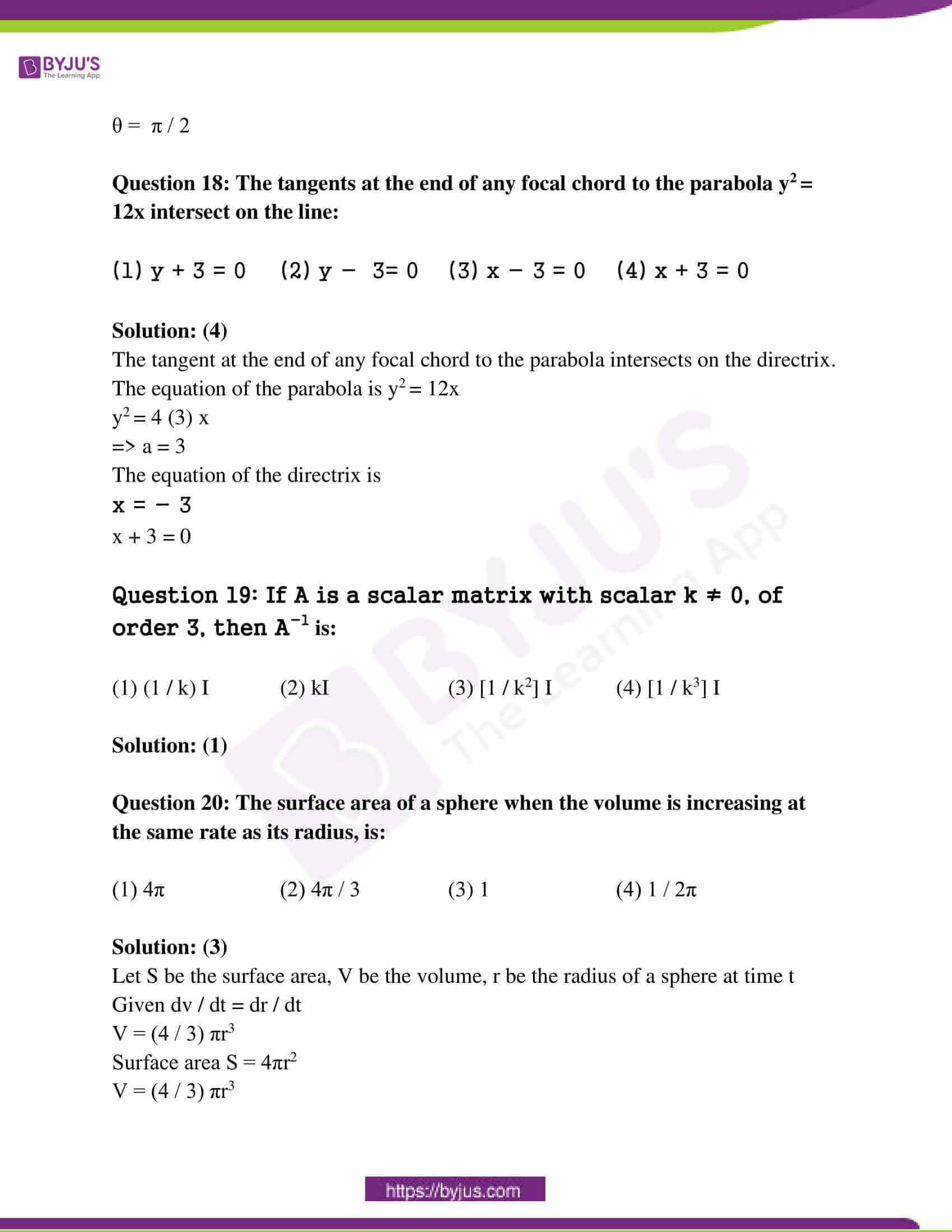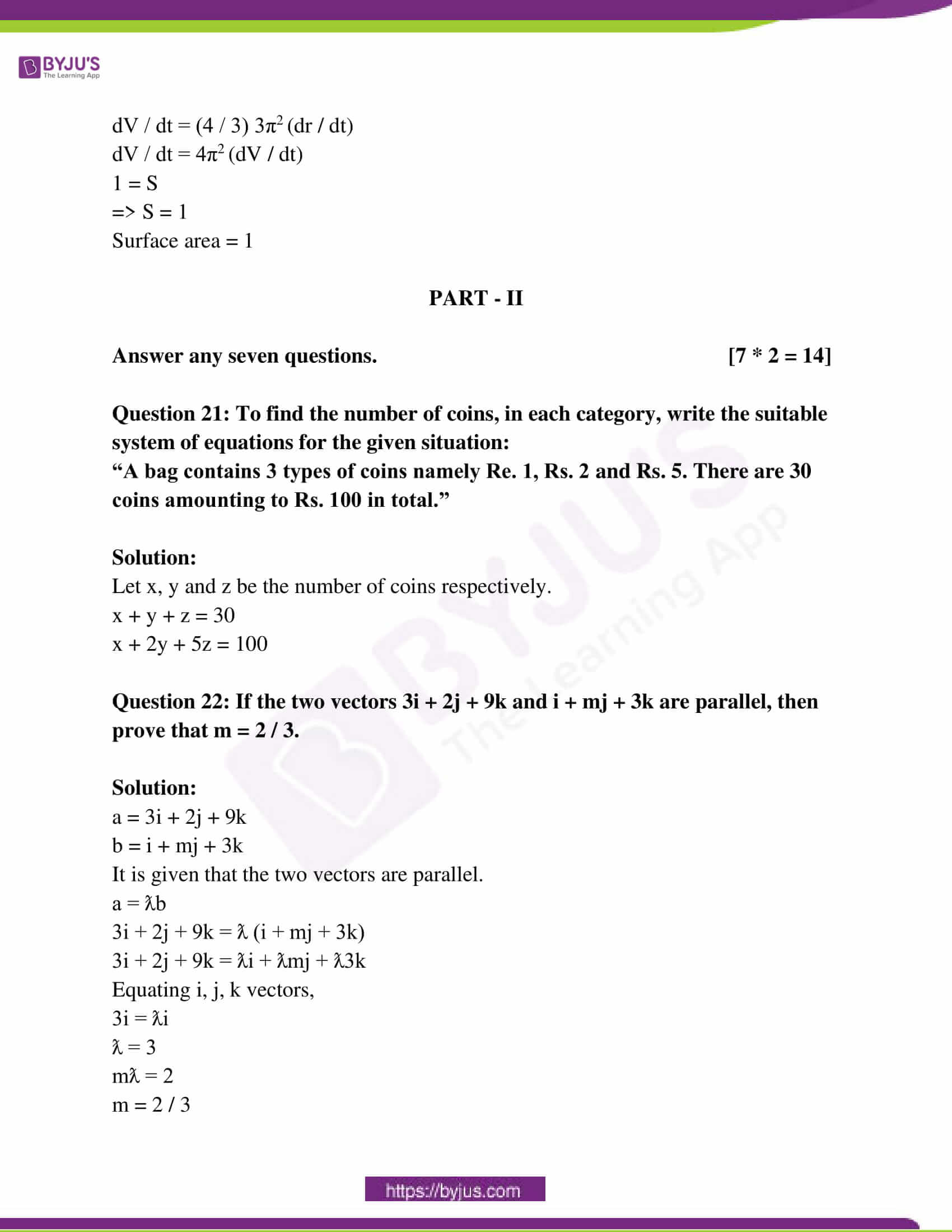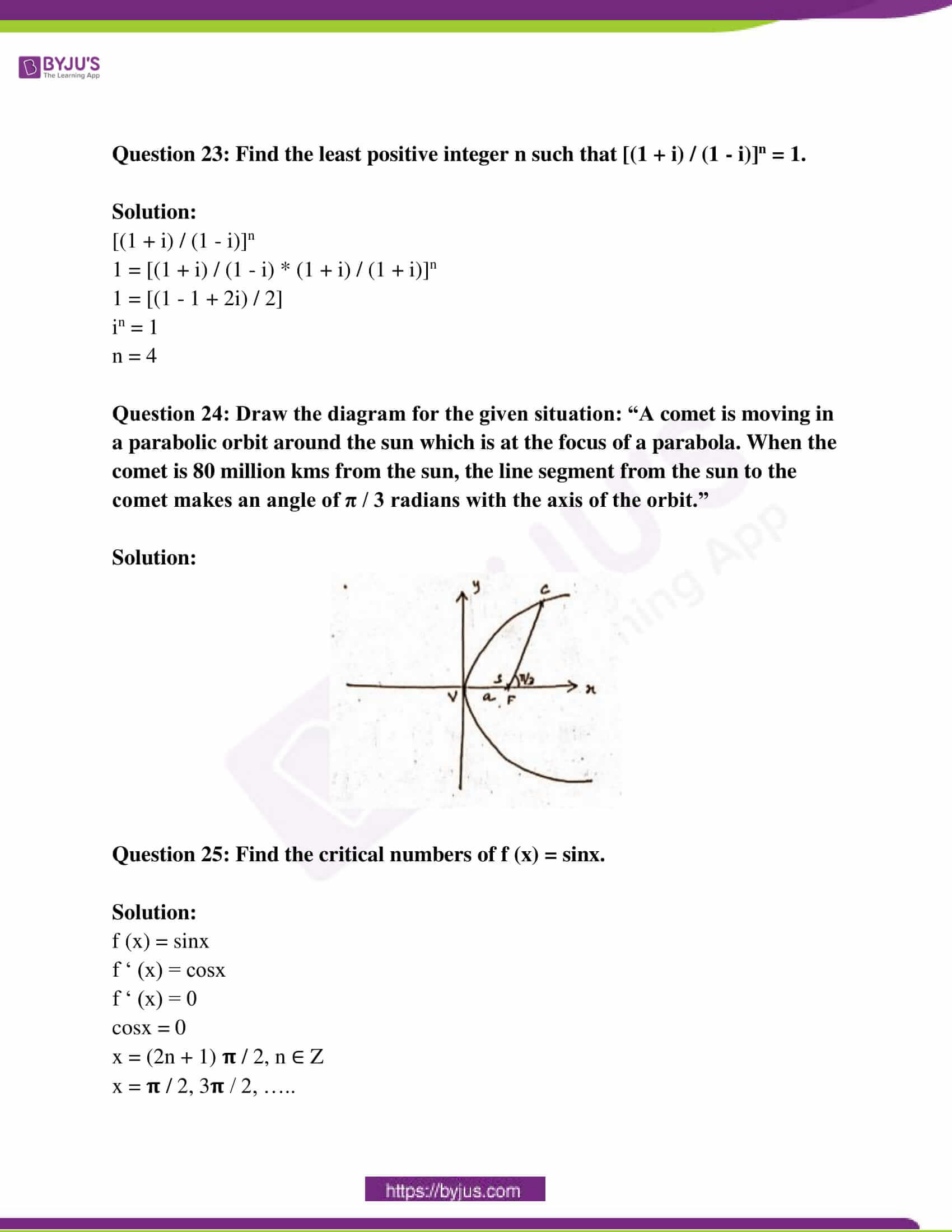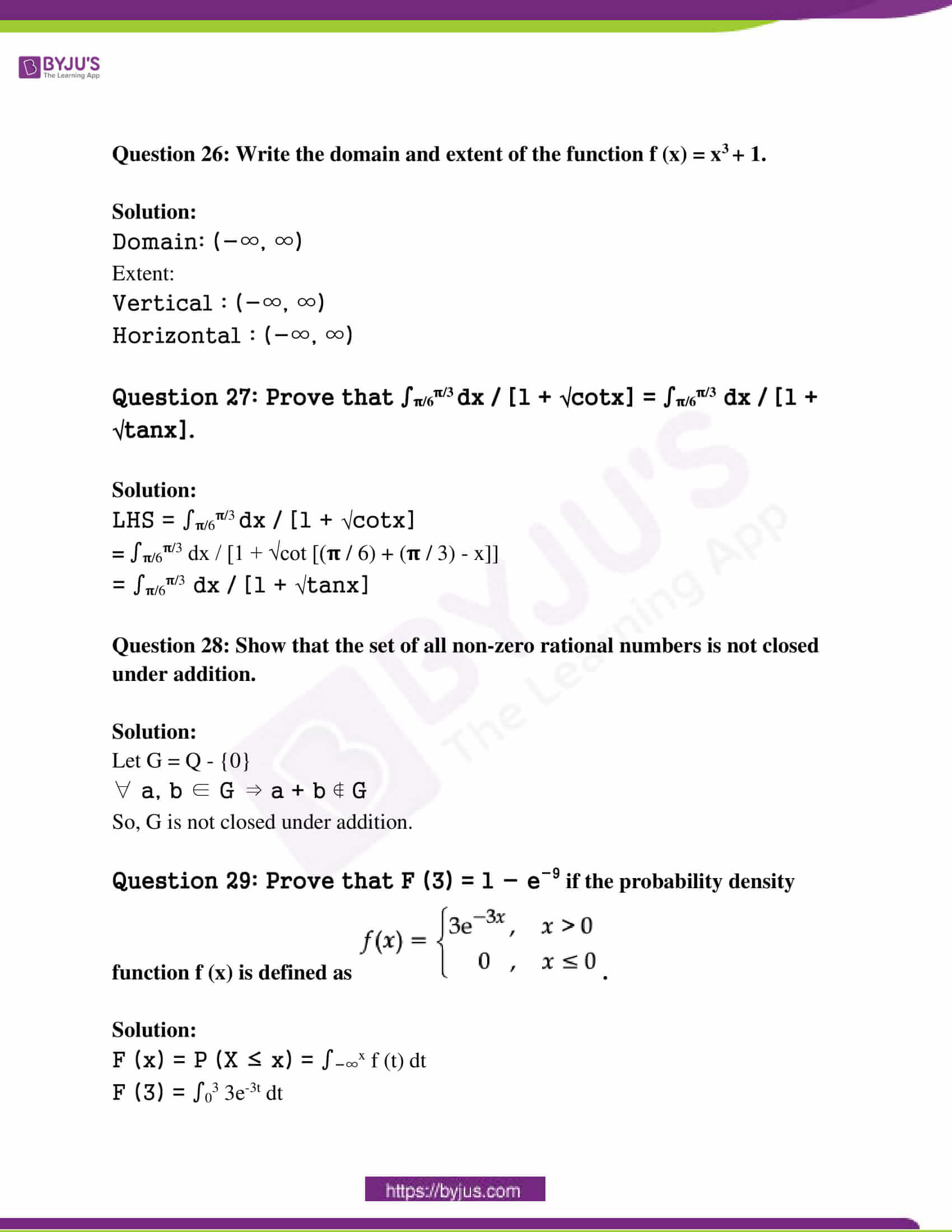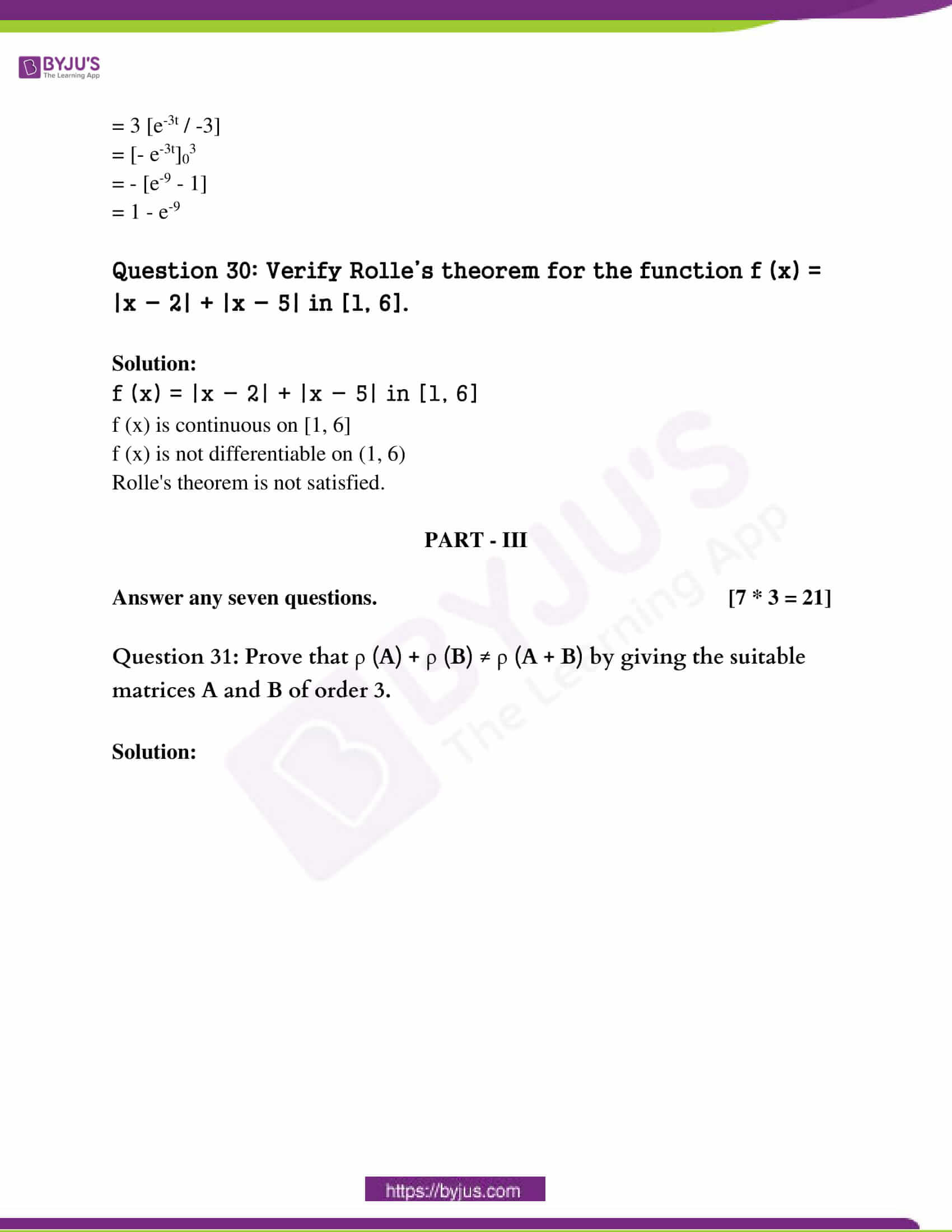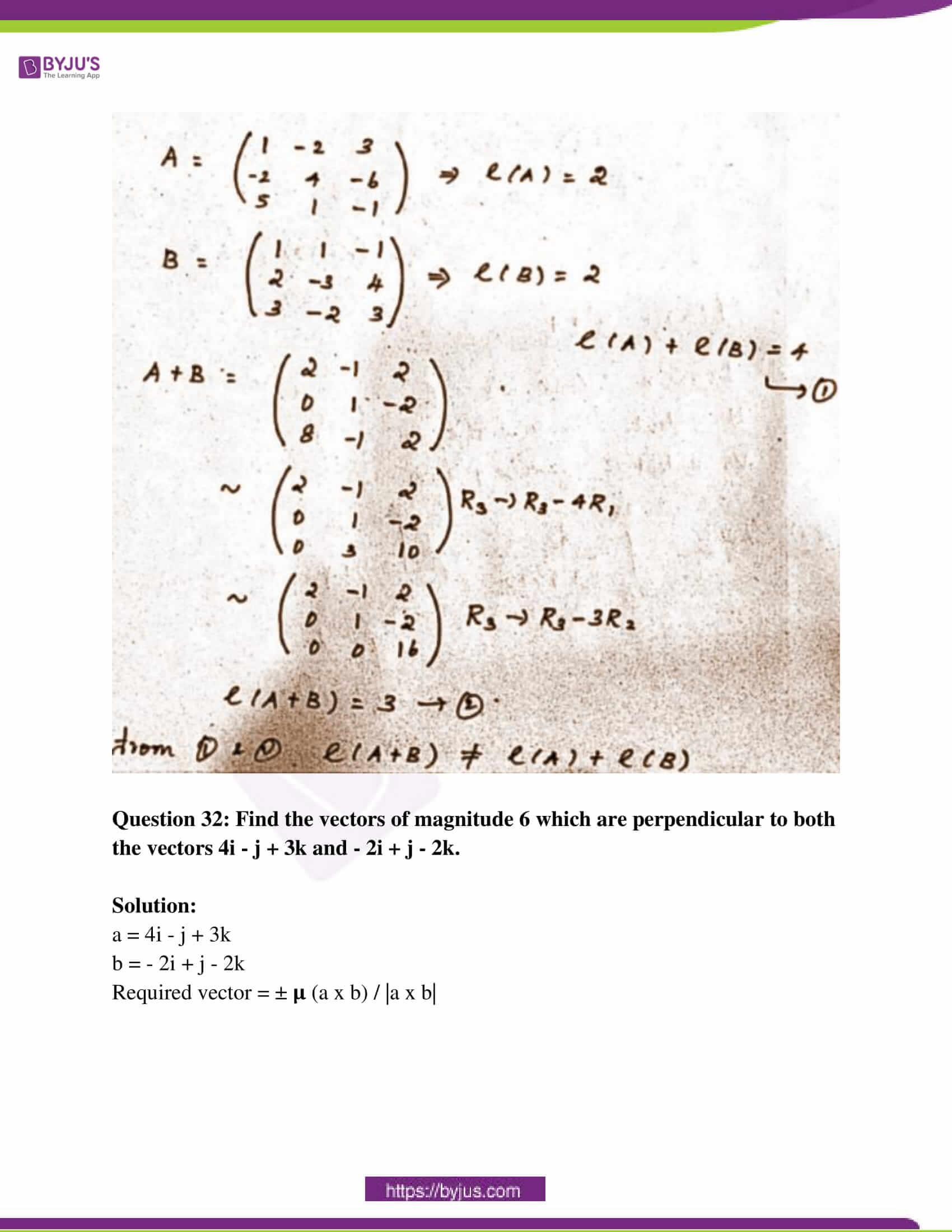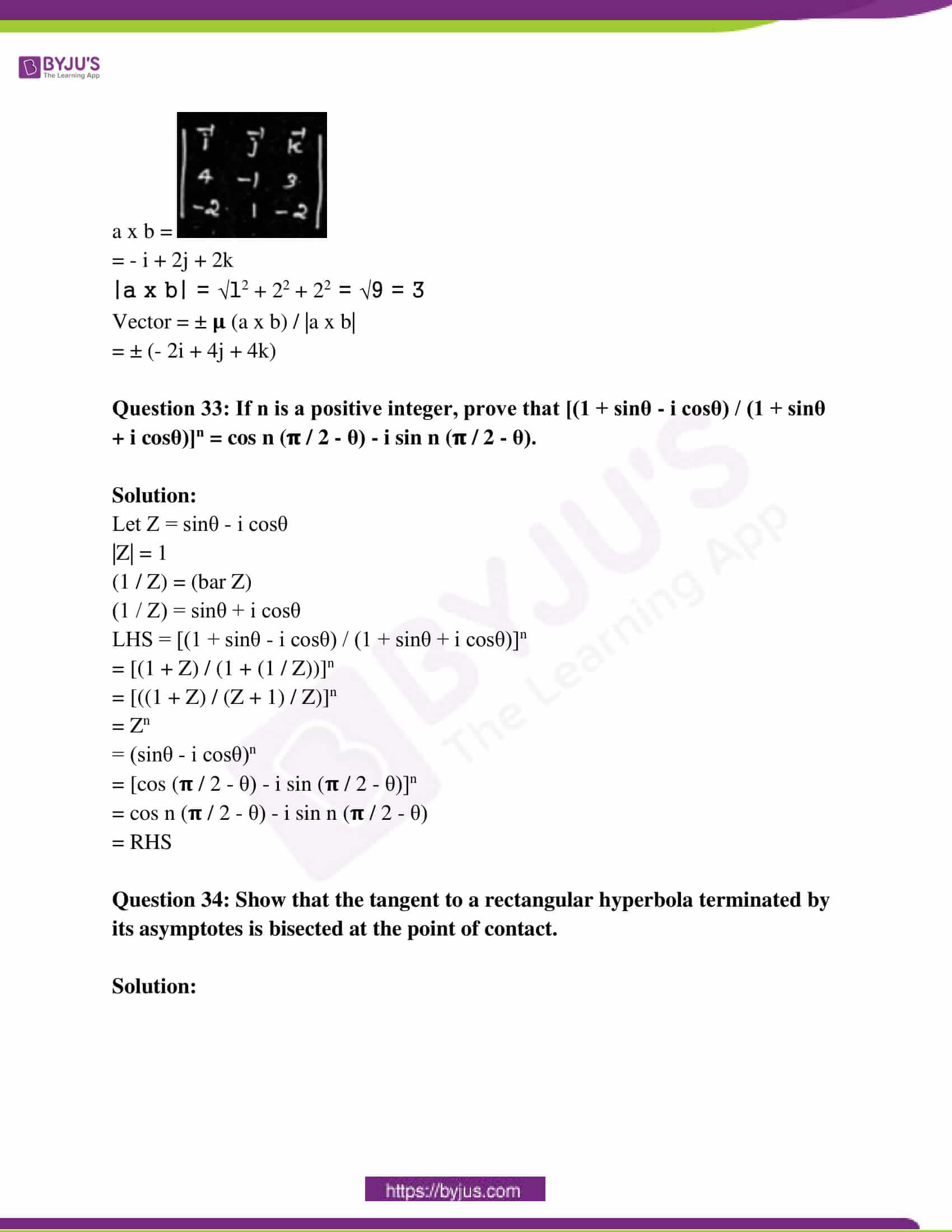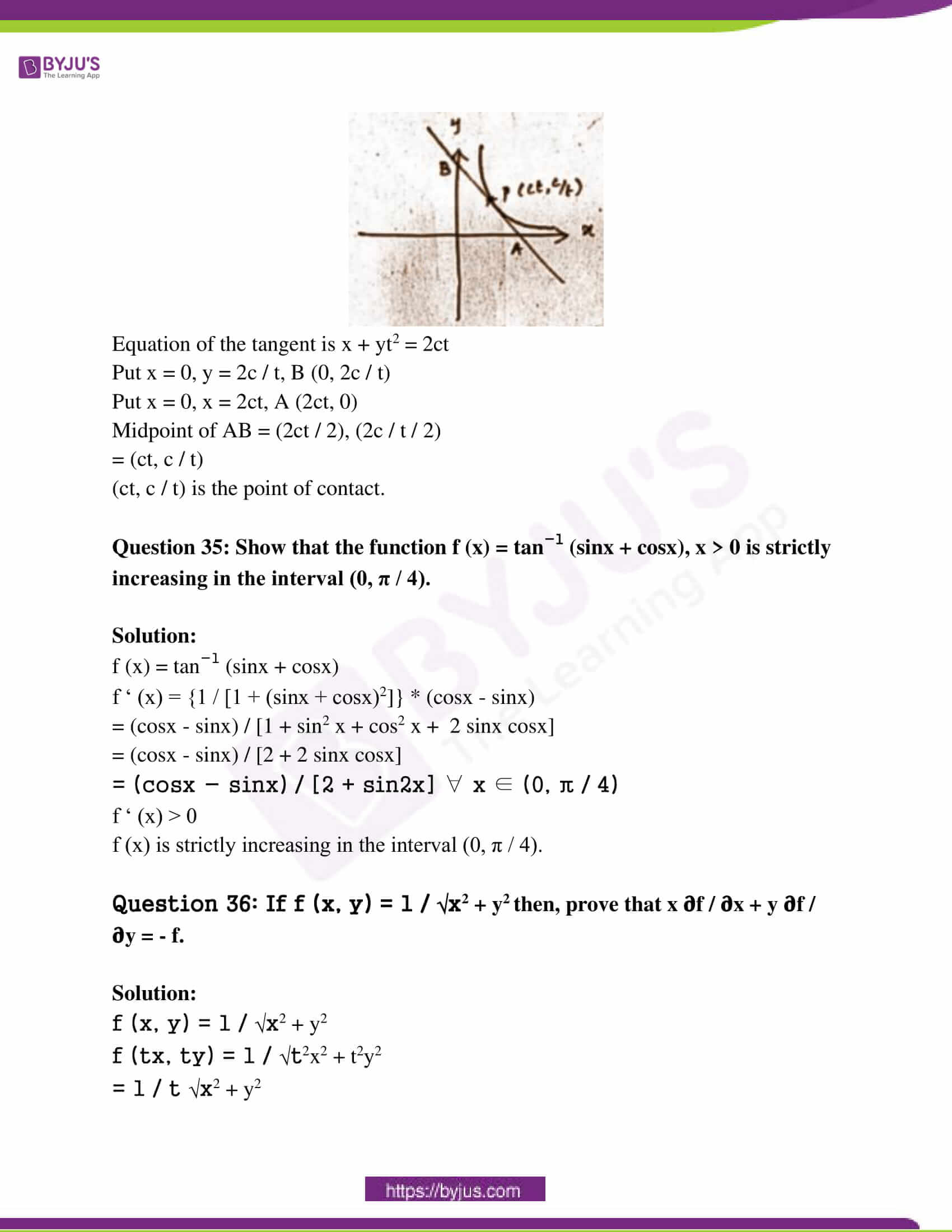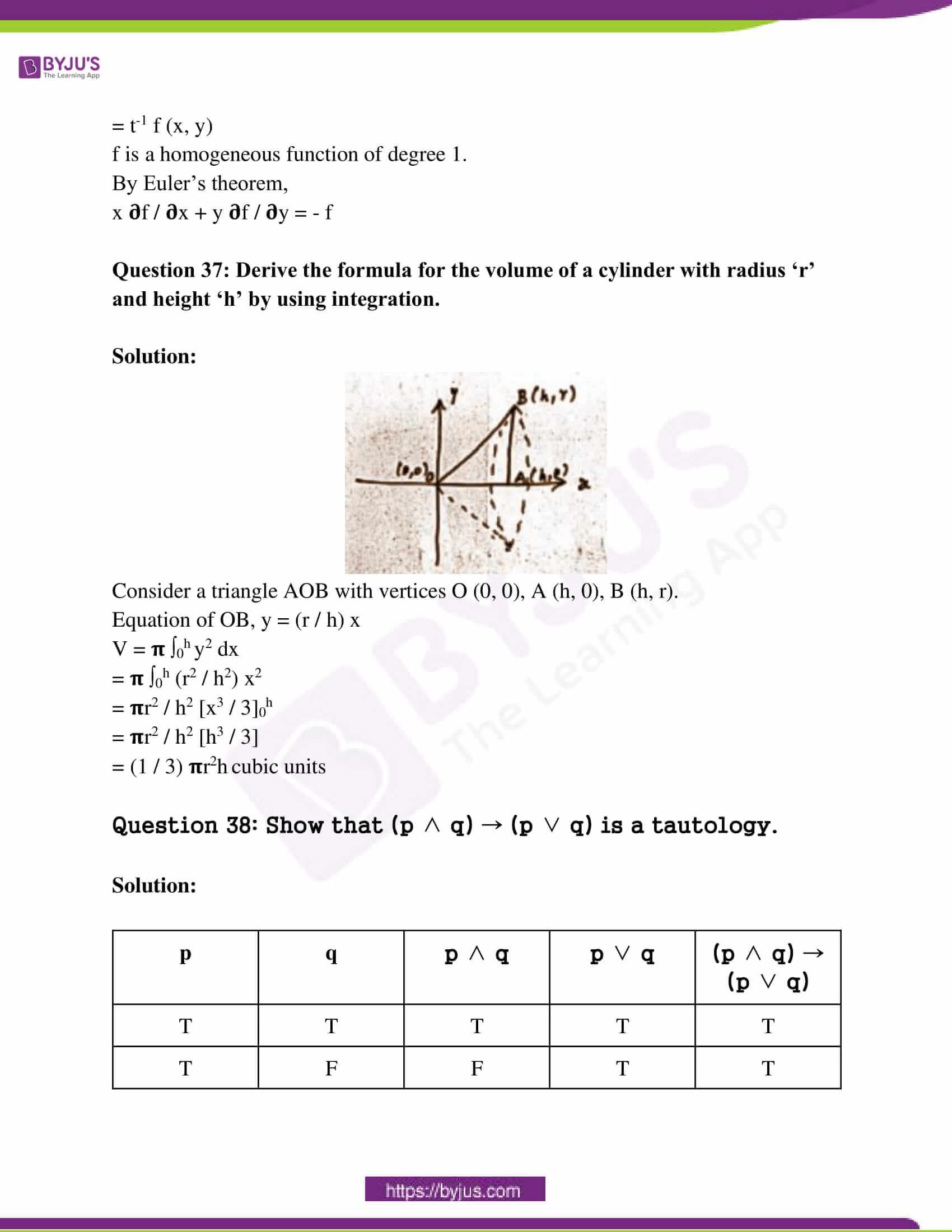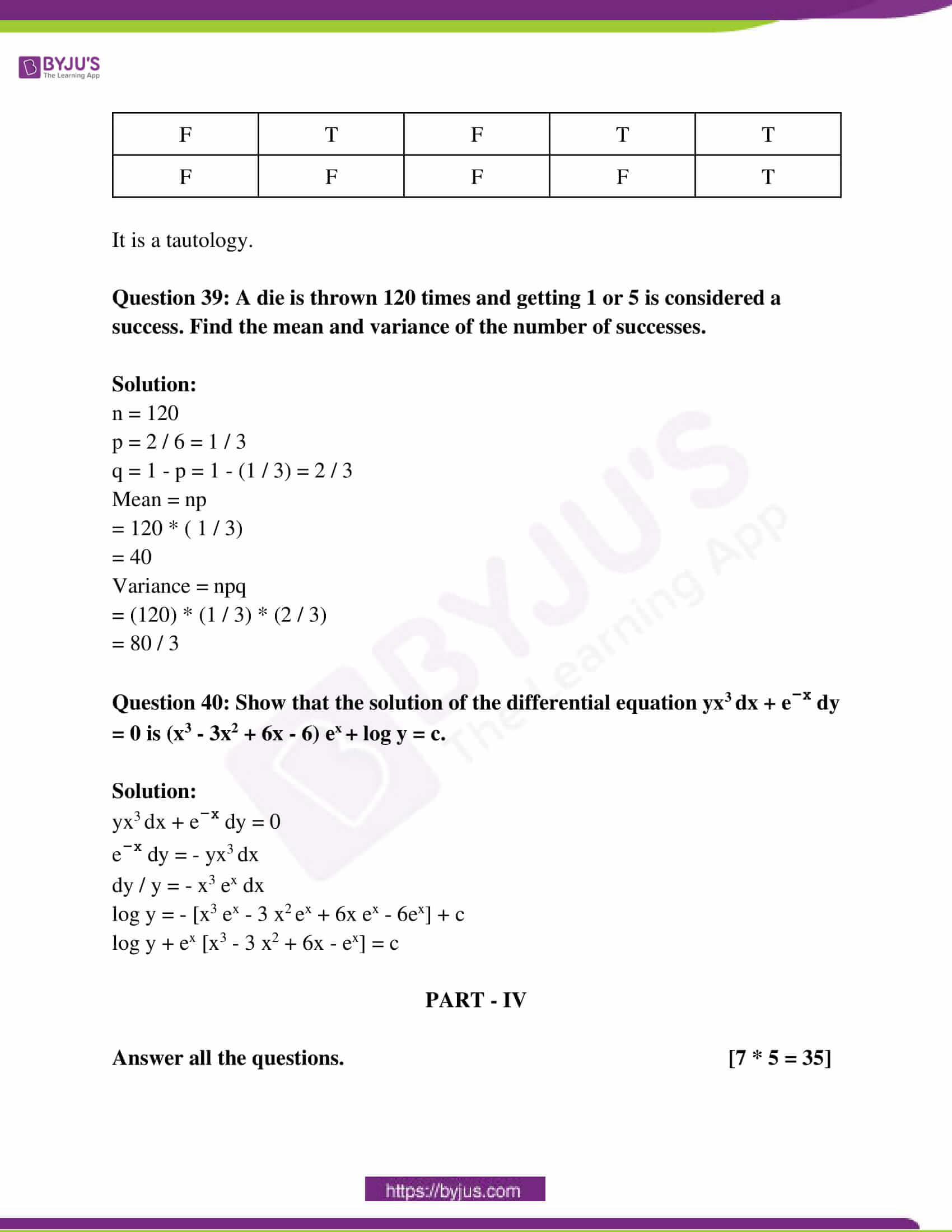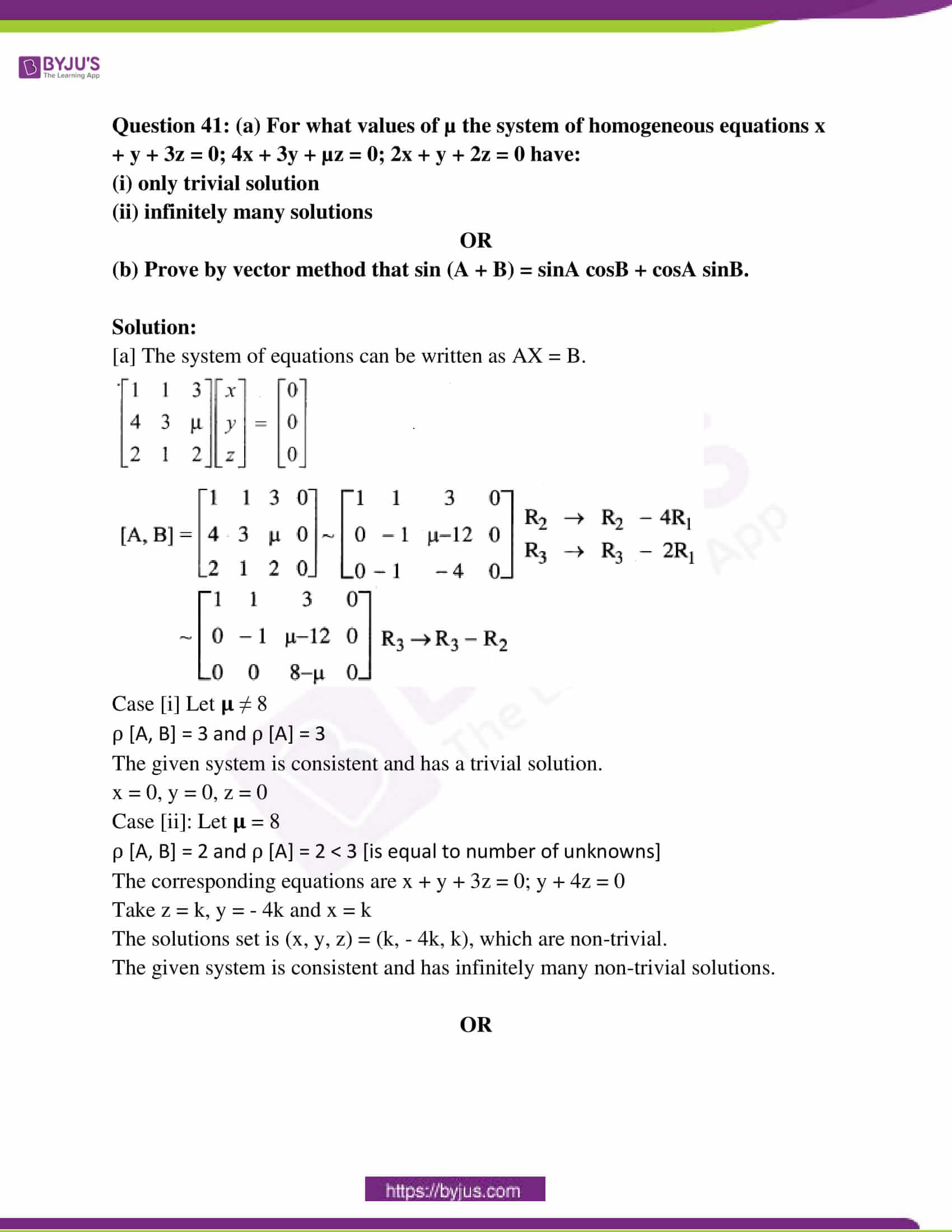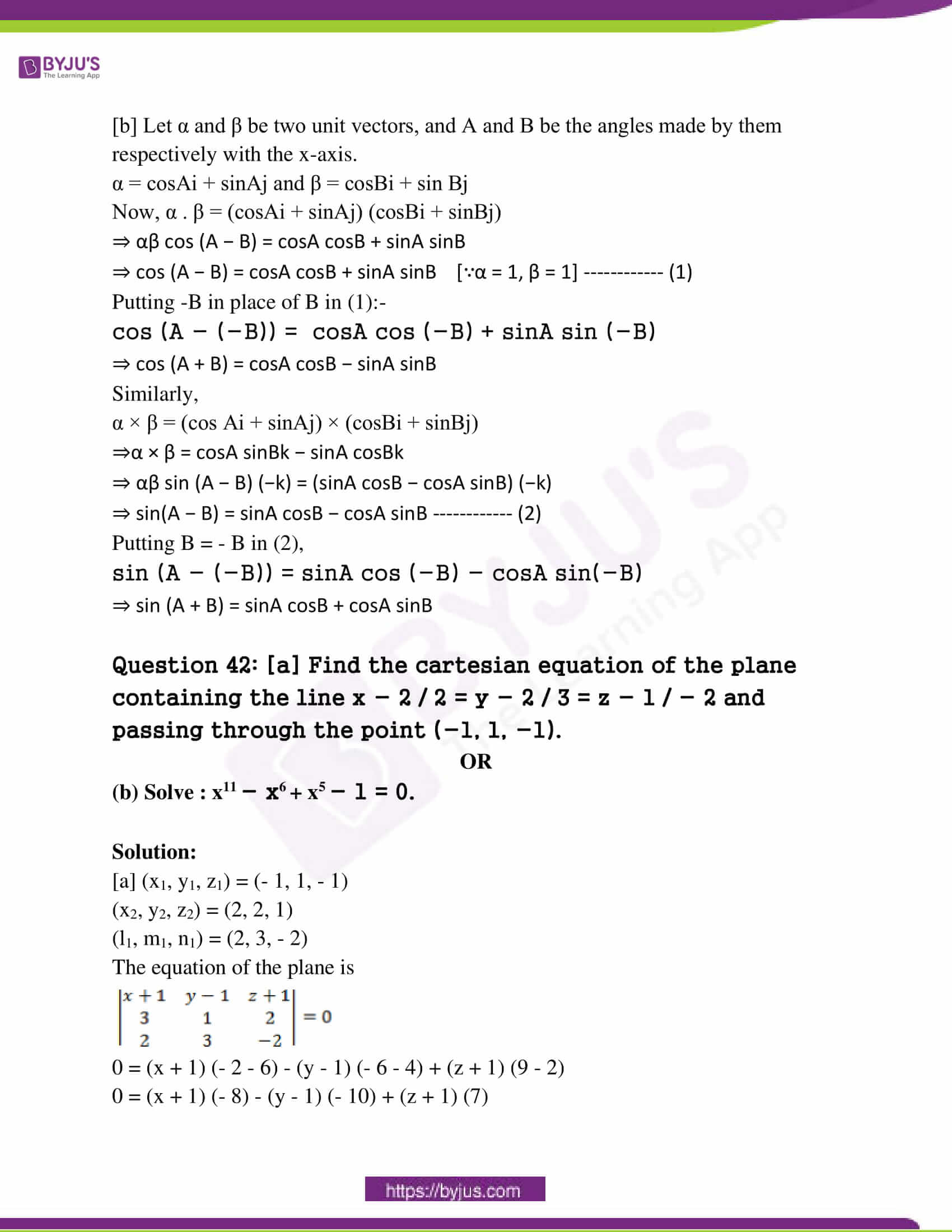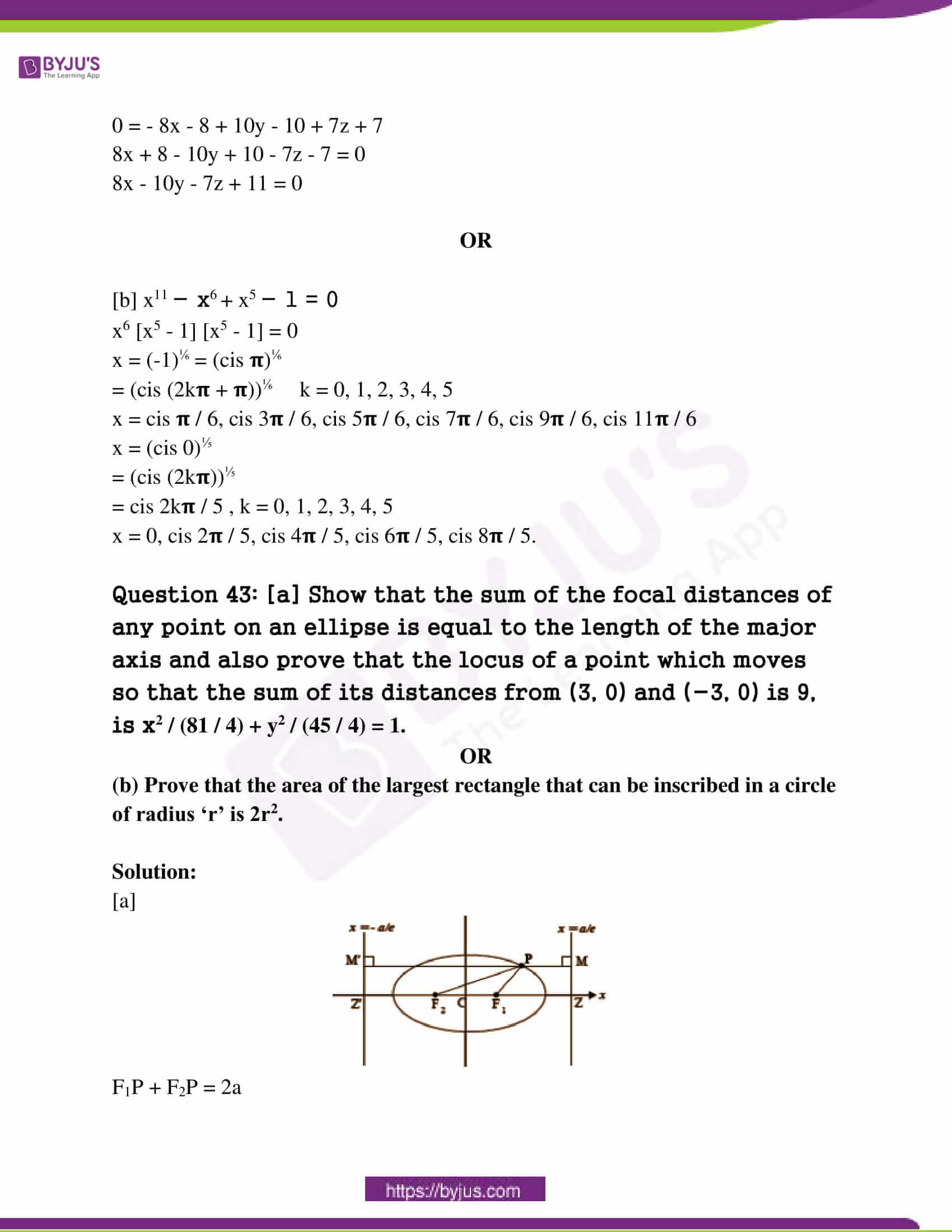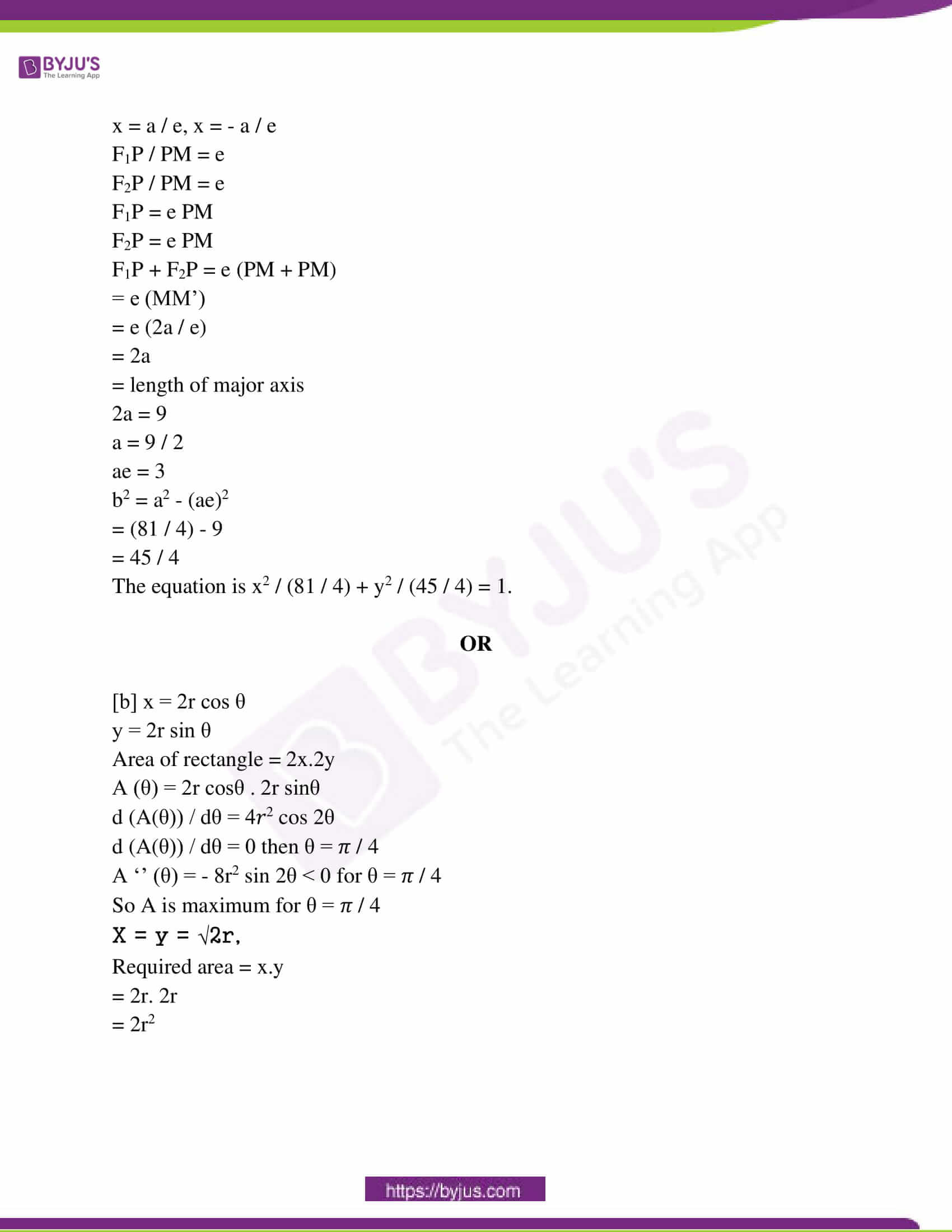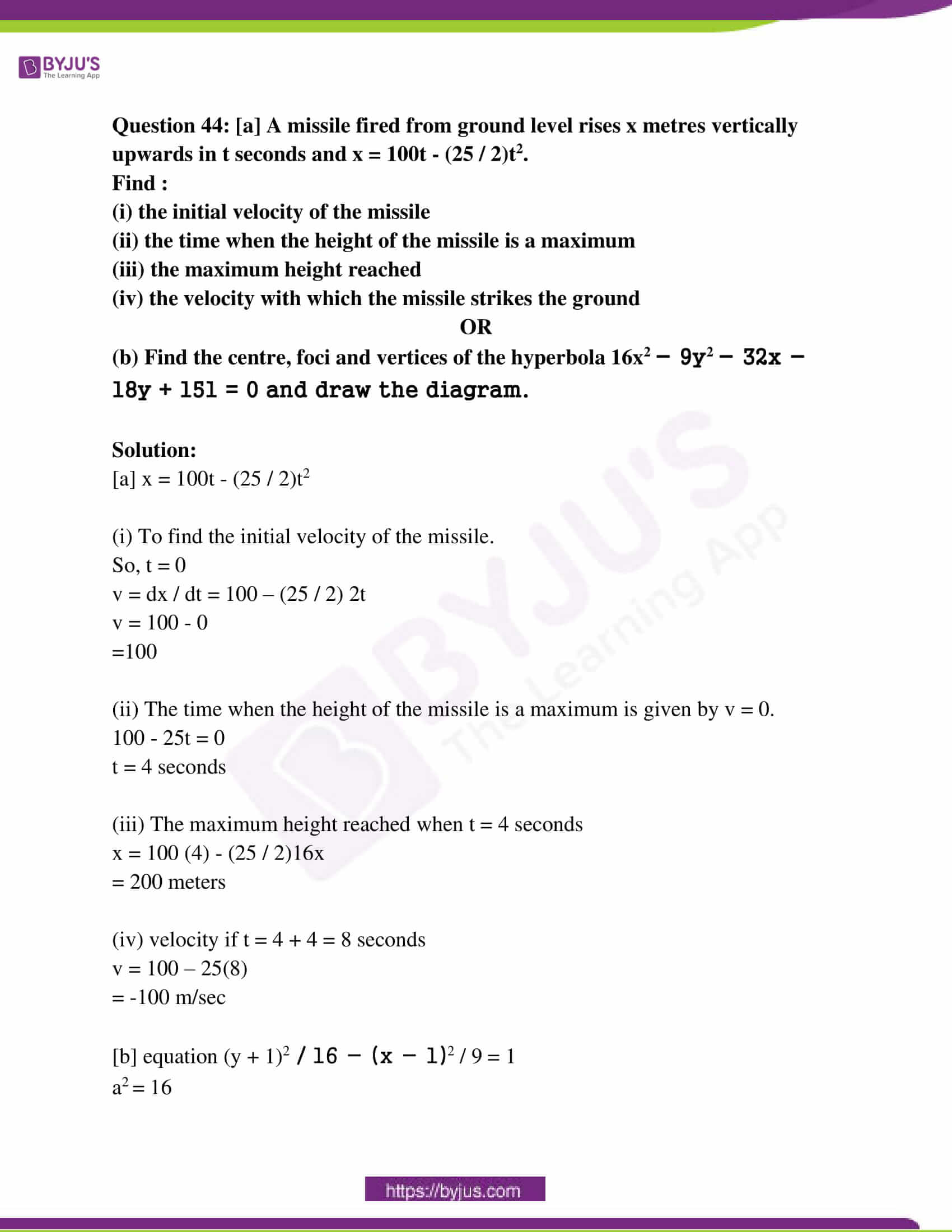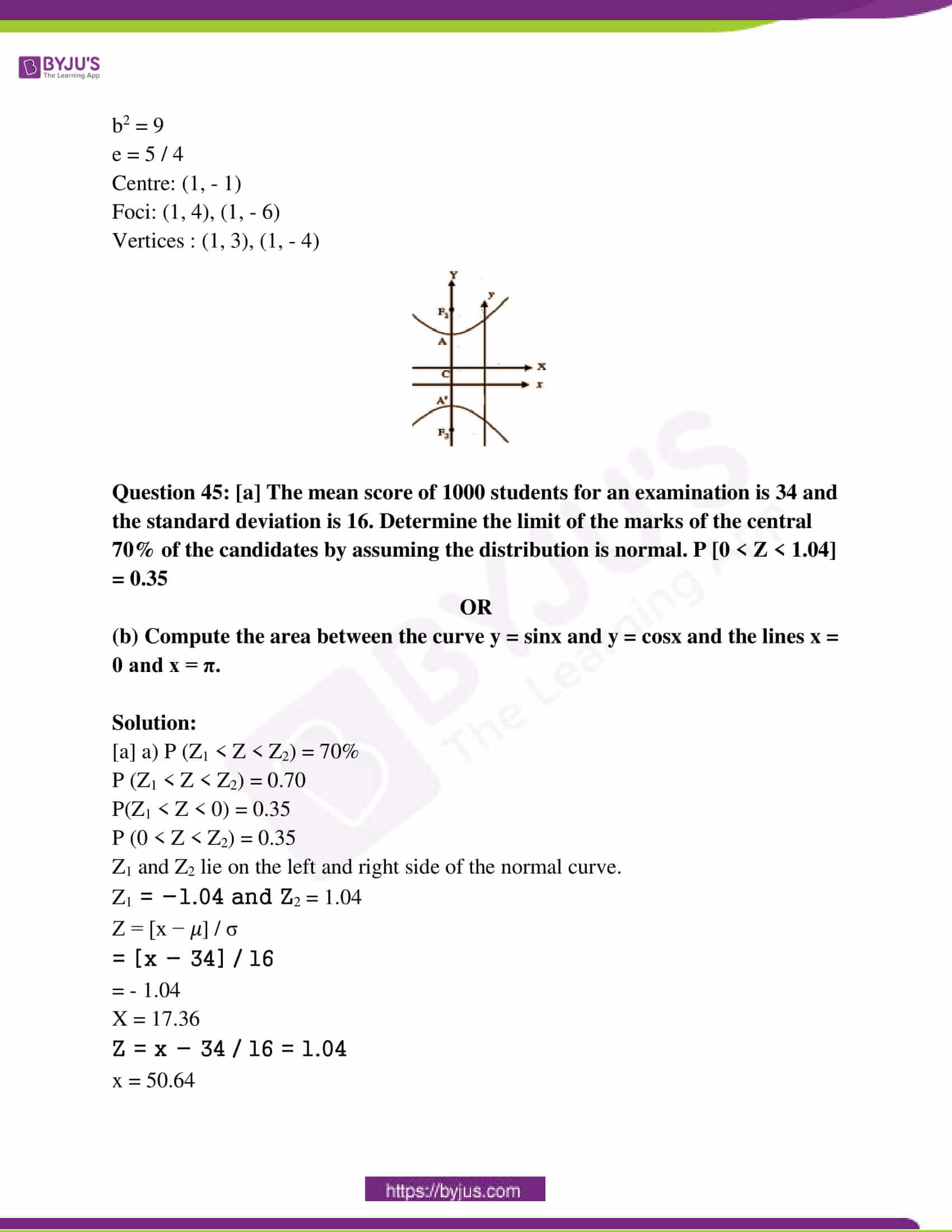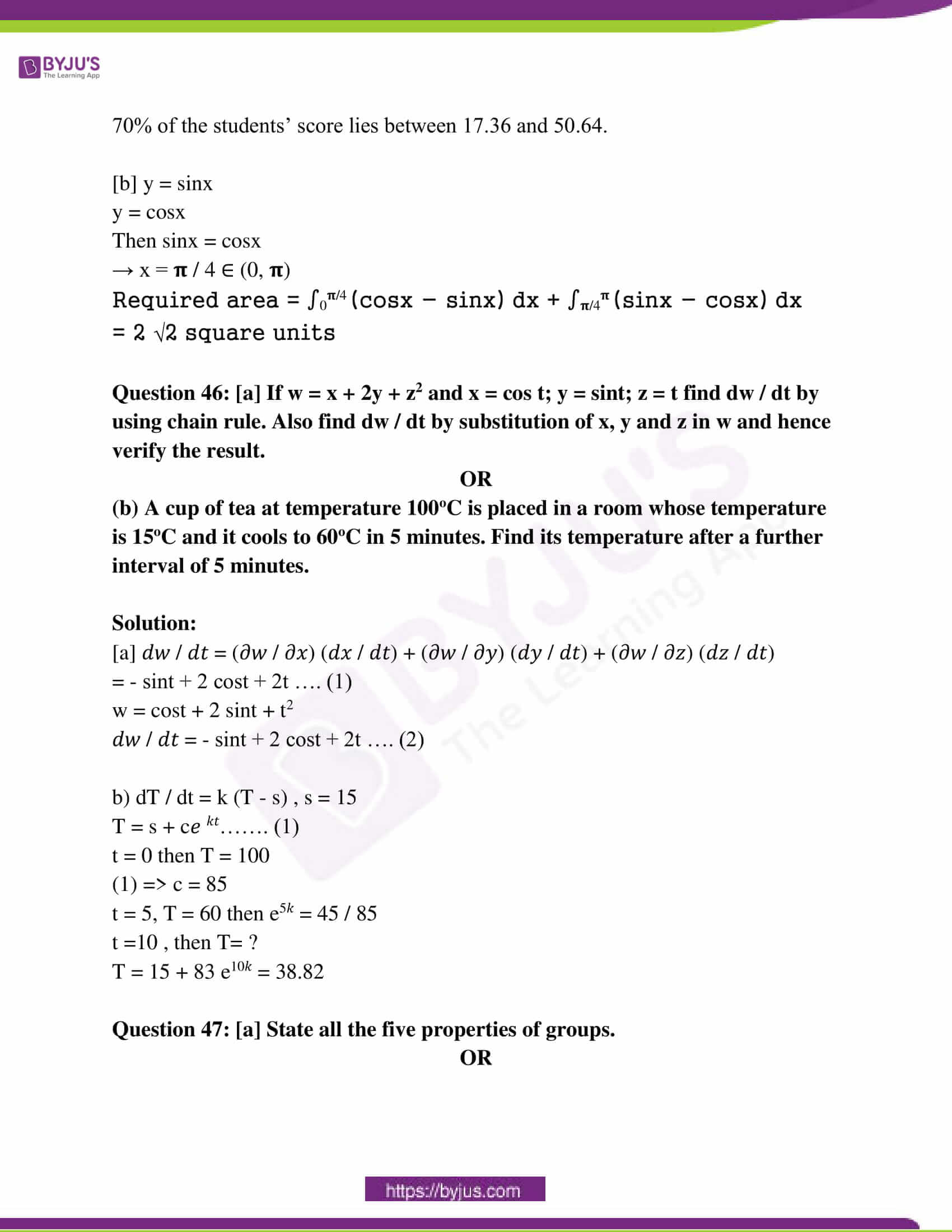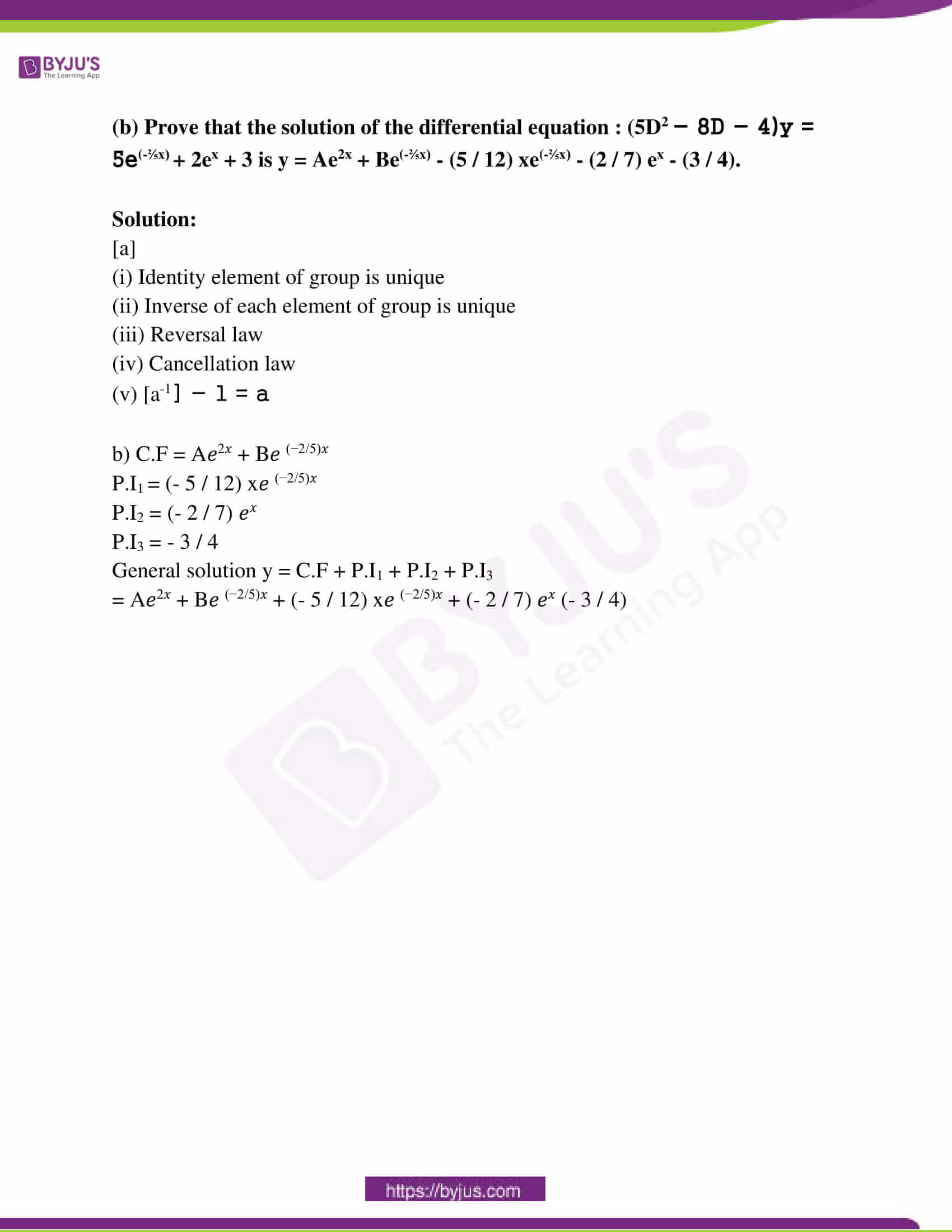PART – I

Choose the most appropriate answer from the given four alternatives and

write the option code and the corresponding answer. [20 * 1 = 20]

Question 1: The percentage error in the 11th root of the number 28 is approximately __________ times the percentage error in 28.

(1) 11 (2) 28 (3) 1 / 28 (4) 1 / 11

Solution: (4)

Let y = x1/11

Taking log on both sides

log y = (1 / 11) log x

dy / y = (1 / 11) (dx / x)

dy / y * 100 = (1 / 11) (dx / x) * 100

The percentage error in x = 28 is dx / x * 100 = 28

The percentage error in y = (dy / y) * 100 = (1 / 11) * 28

Question 2: The line 5x − 2y + 4k = 0 is a tangent to 4x2 − y2 = 36, then k is:

(1) 9 / 4 (2) 81 / 16 (3) 4 / 9 (4) 2 / 3

Solution: (1)

The equation of the hyperbola is

4x2 − y2 = 36

4x2 / 36 − y2 / 36 = 1

4x2 / 9 − y2 / 36 = 1 —- (1)

a2 = 9

b2 = 36

The equation of the line is

5x − 2y + 4k = 0

2y = 5x + 4k

y = 5 / 2 + 2k —- (2)

From equation (2)

m = 5 / 2 c = 2k

c2 = a2 m2 − b2

(2k)2 = 9 (5 / 2)2 − 36

4k2 = 9 × (25 / 4) − 36

4k2 = (225 − 144) / 4

k2 = 81 / 16

=> k = 9 / 4

Question 3: In the multiplicative group of the cube root of unity, the order of ω2 is : [ω is a complex cube root of unity]

(1) 2 (2) 1 (3) 4 (4) 3

Solution: (4)

In the cube root of unity

ω3 = 1

1 + ω + ω2 = 0

2)2 = ω4 = ω3 . ω = ω

2)3 = ω6 = (ω3)2 = (1)2 = 1

0 (ω2) = 3

Question 4: If f (x) and g (x) are two functions as defined in Generalized law of mean then Lagrange’s law of mean is a particular case of Generalised law of mean for:

(1) f ‘ (x) = 0

(2) g ’ (x) = 0

(3) g (x) is an identity function

(4) f (x) is an identity function

Solution: (3)

Question 5: If − x − iy lies in the first quadrant, then − ix + y lies in the:

Solution: (4)

Question 6: Which of the following is a tautology?

(1) p ∨ (~p) (2) p ∧ (~p) (3) p ∨ q (4) p ∧ q

Which of the following is a tautology ? (1) p∨(~p) (2) p∧(~p) (3) p ∨ q (4) p ∧ q

Solution: (1)

Question 7: Variance of the random variable X is 4. Its mean is 2. Then E (X2) is:

(1) 6 (2) 8 (3) 2 (4) 4

Solution: (2)

Variance = E (X2) – (E (X))2

4 = E (X2) – 22

4 + 4 = E (X2)

8 = E (X2)

Question 8: r = si – tk is the equation of :

(1) yz – plane (2) xz – plane

(3) a straight line joining the points i and k (4) xy – plane

Solution: (2)

Question 9: Which one of the following statements is true about the curve y = x1/3?

(1) The curve has a point of inflection in which y’’ does not exist

(2) The curve has more than one point of inflection

(3) The curve has no point of inflection

(4) The curve has a point of inflection in which y’’ = 0

Solution: (1)

Question 10: If z1 = 1 + 2i, z2 = 1 − 3i and z3 = 2 + 4i then, the points on the Argand diagram representing z1 z2 z3, 2 z1 z2 z3, −7 z1 z2 z3 are:

(1) Vertices of an isosceles triangle

(2) Collinear

(3) Vertices of a right-angled triangle

(4) Vertices of an equilateral triangle

Solution: (2)

Question 11: In the homogeneous system ρ(A) is less than the number of unknowns, then the system has:

(1) only non-trivial solutions

(2) no solution

(3) only trivial solution

(4) trivial solution and infinitely many non-trivial solutions

Solution: (4)

Question 12: y = cx − c2 is the general solution of the differential equation:

(1) y’ = c

(2) (y’)2 + xy’ + y = 0

(3) (y’)2 − xy’ + y = 0

(4) y’’ = 0

Solution: (3)

Question 13: The order and degree of the differential equation y’ + (y’’)2 = x (x + y’’)2 are:

(1) 1, 2 (2) 1, 1 (3) 2, 2 (4) 2, 1

Solution: (3)

Question 14: The value of ∫0𝛑/2 [tanx – cotx] / [1 + tanx cotx] is:

(1) π / 4 (2) π (3) π / 2 (4) 0

Solution: (4)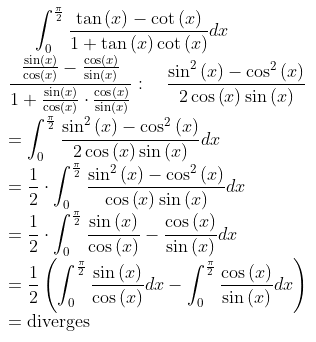Question 15: In a Poisson distribution if P (X = 2) = P (X = 3) then, the value of its parameter λ is:

(1) 3 (2) 0 (3) 6 (4) 2

Solution: (1)

Question 16: The surface area of the solid of revolution of the region bounded by x2 + y2 = 4, x = −2 and x = 2 about x-axis is:

(1) 64π (2) 32π (3) 8π (4) 16π

Solution: (4)

Question 17: If a + b + c = 0, |a| = 3, |b| = 4, |c| = 5 then, the angle between a and b is:

(1) 5π / 3 (2) π / 2 (3) π / 6 (4) 2π / 3

Solution: (2)

a + b + c = 0

|a| = 3

|b| = 4

|c| = 5

θ = cos-1 [ (a . b) / |a| |b| ]

a + b + c = 0

a + b = – c

(a + b)2 = (-c)2

|a|2 + |b|2 + 2ab = |c|2

32 + 42 + 2ab = 52

9 + 16 + 2ab = 25

2ab = 0

ab = 0

θ = cos-1 [0 / 3 . 4]

θ = cos-1 

θ = π / 2

Question 18: The tangents at the end of any focal chord to the parabola y2 = 12x intersect on the line:

(1) y + 3 = 0 (2) y − 3= 0 (3) x − 3 = 0 (4) x + 3 = 0

Solution: (4)

The tangent at the end of any focal chord to the parabola intersects on the directrix.

The equation of the parabola is y2 = 12x

y2 = 4 (3) x

=> a = 3

The equation of the directrix is

x = − 3

x + 3 = 0

Question 19: If A is a scalar matrix with scalar k ≠ 0, of order 3, then A−1 is:

(1) (1 / k) I (2) kI (3) [1 / k2] I (4) [1 / k3] I

Solution: (1)

Question 20: The surface area of a sphere when the volume is increasing at the same rate as its radius, is:

(1) 4π (2) 4π / 3 (3) 1 (4) 1 / 2π

Solution: (3)

Let S be the surface area, V be the volume, r be the radius of a sphere at time t

Given dv / dt = dr / dt

V = (4 / 3) πr3

Surface area S = 4πr2

V = (4 / 3) πr3

dV / dt = (4 / 3) 3π2 (dr / dt)

dV / dt = 4π2 (dV / dt)

1 = S

=> S = 1

Surface area = 1

PART – II

Answer any seven questions. [7 * 2 = 14]

Question 21: To find the number of coins, in each category, write the suitable system of equations for the given situation:

“A bag contains 3 types of coins namely Re. 1, Rs. 2 and Rs. 5. There are 30 coins amounting to Rs. 100 in total.”

Solution:

Let x, y and z be the number of coins respectively.

x + y + z = 30

x + 2y + 5z = 100

Question 22: If the two vectors 3i + 2j + 9k and i + mj + 3k are parallel, then prove that m = 2 / 3.

Solution:

a = 3i + 2j + 9k

b = i + mj + 3k

It is given that the two vectors are parallel.

a = ƛb

3i + 2j + 9k = ƛ (i + mj + 3k)

3i + 2j + 9k = ƛi + ƛmj + ƛ3k

Equating i, j, k vectors,

3i = ƛi

ƛ = 3

mƛ = 2

m = 2 / 3

Question 23: Find the least positive integer n such that [(1 + i) / (1 – i)]n = 1.

Solution:

[(1 + i) / (1 – i)]n

1 = [(1 + i) / (1 – i) * (1 + i) / (1 + i)]n

1 = [(1 – 1 + 2i) / 2]

in = 1

n = 4

Question 24: Draw the diagram for the given situation: “A comet is moving in a parabolic orbit around the sun which is at the focus of a parabola. When the comet is 80 million kms from the sun, the line segment from the sun to the comet makes an angle of π / 3 radians with the axis of the orbit.”

Solution: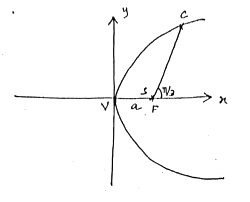Question 25: Find the critical numbers of f (x) = sinx.

Solution:

f (x) = sinx

f ‘ (x) = cosx

f ‘ (x) = 0

cosx = 0

x = (2n + 1) 𝛑 / 2, n ∈ Z

x = 𝛑 / 2, 3𝛑 / 2, …..

Question 26: Write the domain and extent of the function f (x) = x3 + 1.

Solution:

Domain: (−∞, ∞)

Extent:

Vertical : (−∞, ∞)

Horizontal : (−∞, ∞)

Question 27: Prove that ∫𝛑/6𝛑/3 dx / [1 + √cotx] = ∫𝛑/6𝛑/3 dx / [1 + √tanx].

Solution:

LHS = ∫𝛑/6𝛑/3 dx / [1 + √cotx]

= 𝛑/6𝛑/3 dx / [1 + √cot [(𝛑 / 6) + (𝛑 / 3) – x]]

= ∫𝛑/6𝛑/3 dx / [1 + √tanx]

Question 28: Show that the set of all non-zero rational numbers is not closed under addition.

Solution:

Let G = Q – {0}

∀ a, b ∈ G ⇒ a + b ∉ G

So, G is not closed under addition.

Question 29: Prove that F (3) = 1 − e−9 if the probability density function f (x) is defined as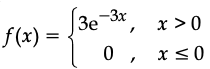.

Solution:

F (x) = P (X ≤ x) = ∫-∞x f (t) dt

F (3) = ∫03 3e-3t dt

= 3 [e-3t / -3]

= [- e-3t]03

= – [e-9 – 1]

= 1 – e-9

Question 30: Verify Rolle’s theorem for the function f (x) = |x − 2| + |x − 5| in [1, 6].

Solution:

f (x) = |x − 2| + |x − 5| in [1, 6]

f (x) is continuous on [1, 6]

f (x) is not differentiable on (1, 6)

Rolle’s theorem is not satisfied.

PART – III

Answer any seven questions. [7 * 3 = 21]

Question 31: Prove that ρ (A) + ρ (B) ≠ ρ (A + B) by giving the suitable matrices A and B of order 3.

Solution: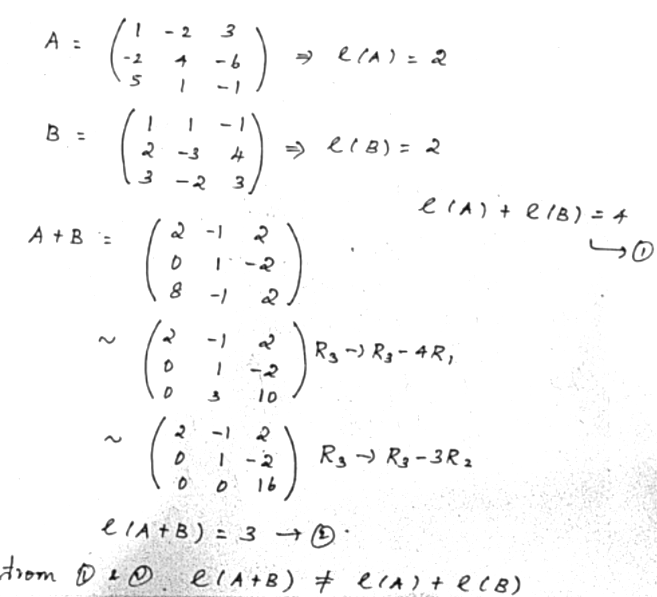Question 32: Find the vectors of magnitude 6 which are perpendicular to both the vectors 4i – j + 3k and – 2i + j – 2k.

Solution:

a = 4i – j + 3k

b = – 2i + j – 2k

Required vector = ± 𝛍 (a x b) / |a x b|

a x b =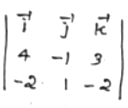= – i + 2j + 2k

|a x b| = √12 + 22 + 22 = √9 = 3

Vector = ± 𝛍 (a x b) / |a x b|

= ± (- 2i + 4j + 4k)

Question 33: If n is a positive integer, prove that [(1 + sinθ – i cosθ) / (1 + sinθ + i cosθ)]n = cos n (𝛑 / 2 – θ) – i sin n (𝛑 / 2 – θ).

Solution:

Let Z = sinθ – i cosθ

|Z| = 1

(1 / Z) = (bar Z)

(1 / Z) = sinθ + i cosθ

LHS = [(1 + sinθ – i cosθ) / (1 + sinθ + i cosθ)]n

= [(1 + Z) / (1 + (1 / Z))]n

= [((1 + Z) / (Z + 1) / Z)]n

= Zn

= (sinθ – i cosθ)n

= [cos (𝛑 / 2 – θ) – i sin (𝛑 / 2 – θ)]n

= cos n (𝛑 / 2 – θ) – i sin n (𝛑 / 2 – θ)

= RHS

Question 34: Show that the tangent to a rectangular hyperbola terminated by its asymptotes is bisected at the point of contact.

Solution: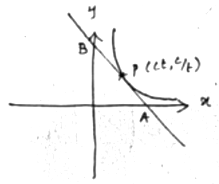Equation of the tangent is x + yt2 = 2ct

Put x = 0, y = 2c / t, B (0, 2c / t)

Put x = 0, x = 2ct, A (2ct, 0)

Midpoint of AB = (2ct / 2), (2c / t / 2)

= (ct, c / t)

(ct, c / t) is the point of contact.

Question 35: Show that the function f (x) = tan−1 (sinx + cosx), x > 0 is strictly increasing in the interval (0, π / 4).

Solution:

f (x) = tan−1 (sinx + cosx)

f ‘ (x) = {1 / [1 + (sinx + cosx)2]} * (cosx – sinx)

= (cosx – sinx) / [1 + sin2 x + cos2 x + 2 sinx cosx]

= (cosx – sinx) / [2 + 2 sinx cosx]

= (cosx – sinx) / [2 + sin2x] ∀ x ∈ (0, π / 4)

f ‘ (x) > 0

f (x) is strictly increasing in the interval (0, π / 4).

Question 36: If f (x, y) = 1 / √x2 + y2 then, prove that x 𝛛f / 𝛛x + y 𝛛f / 𝛛y = – f.

Solution:

f (x, y) = 1 / √x2 + y2

f (tx, ty) = 1 / √t2x2 + t2y2

= 1 / t √x2 + y2

= t-1 f (x, y)

f is a homogeneous function of degree 1.

By Euler’s theorem,

x 𝛛f / 𝛛x + y 𝛛f / 𝛛y = – f

Question 37: Derive the formula for the volume of a cylinder with radius ‘r’ and height ‘h’ by using integration.

Solution: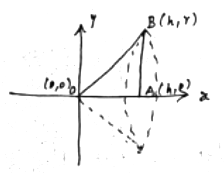Consider a triangle AOB with vertices O (0, 0), A (h, 0), B (h, r).

Equation of OB, y = (r / h) x

V = 𝛑 ∫0h y2 dx

= 𝛑 ∫0h (r2 / h2) x2

= 𝛑r2 / h2 [x3 / 3]0h

= 𝛑r2 / h2 [h3 / 3]

= (1 / 3) 𝛑r2h cubic units

Question 38: Show that (p ∧ q) → (p ∨ q) is a tautology.

Solution:

 p q p ∧ q p ∨ q (p ∧ q) → (p ∨ q) T T T T T T F F T T F T F T T F F F F T

It is a tautology.

Question 39: A die is thrown 120 times and getting 1 or 5 is considered a success. Find the mean and variance of the number of successes.

Solution:

n = 120

p = 2 / 6 = 1 / 3

q = 1 – p = 1 – (1 / 3) = 2 / 3

Mean = np

= 120 * ( 1 / 3)

= 40

Variance = npq

= (120) * (1 / 3) * (2 / 3)

= 80 / 3

Question 40: Show that the solution of the differential equation yx3 dx + e−x dy = 0 is (x3 – 3x2 + 6x – 6) ex + log y = c.

Solution:

yx3 dx + e−x dy = 0

e−x dy = – yx3 dx

dy / y = – x3 ex dx

log y = – [x3 ex – 3 x2 ex + 6x ex – 6ex] + c

log y + ex [x3 – 3 x2 + 6x – ex] = c

PART – IV

Answer all the questions. [7 * 5 = 35]

Question 41: (a) For what values of µ the system of homogeneous equations x + y + 3z = 0; 4x + 3y + µz = 0; 2x + y + 2z = 0 have:

(i) only trivial solution

(ii) infinitely many solutions

OR

(b) Prove by vector method that sin (A + B) = sinA cosB + cosA sinB.

Solution:

[a] The system of equations can be written as AX = B.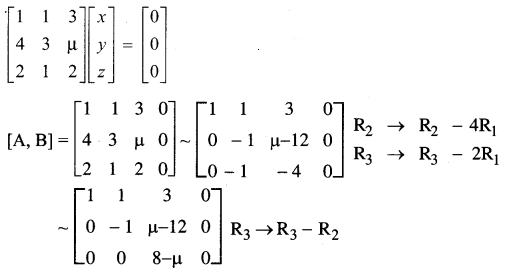Case [i] Let 𝛍 ≠ 8

⍴ [A, B] = 3 and ⍴ [A] = 3

The given system is consistent and has a trivial solution.

x = 0, y = 0, z = 0

Case [ii]: Let 𝛍 = 8

⍴ [A, B] = 2 and ⍴ [A] = 2 < 3 [is equal to number of unknowns]

The corresponding equations are x + y + 3z = 0; y + 4z = 0

Take z = k, y = – 4k and x = k

The solutions set is (x, y, z) = (k, – 4k, k), which are non-trivial.

The given system is consistent and has infinitely many non-trivial solutions.

OR

[b] Let α and β be two unit vectors, and A and B be the angles made by them respectively with the x-axis.

α = cosAi + sinAj and β = cosBi + sin Bj

Now, α . β = (cosAi + sinAj) (cosBi + sinBj)

⇒ αβ cos (A − B) = cosA cosB + sinA sinB

⇒ cos (A − B) = cosA cosB + sinA sinB [∵α = 1, β = 1] ———— (1)

Putting -B in place of B in (1):-

cos (A − (−B)) = cosA cos (−B) + sinA sin (−B)

⇒ cos (A + B) = cosA cosB − sinA sinB

Similarly,

α × β = (cos Ai + sinAj) × (cosBi + sinBj)

⇒α × β = cosA sinBk − sinA cosBk

⇒ αβ sin (A − B) (−k) = (sinA cosB − cosA sinB) (−k)

⇒ sin(A − B) = sinA cosB − cosA sinB ———— (2)

Putting B = – B in (2),

sin (A − (−B)) = sinA cos (−B) − cosA sin(−B)

⇒ sin (A + B) = sinA cosB + cosA sinB

Question 42: [a] Find the cartesian equation of the plane containing the line x – 2 / 2 = y – 2 / 3 = z – 1 / – 2 and passing through the point (−1, 1, −1).

OR

(b) Solve : x11 − x6 + x5 − 1 = 0.

Solution:

[a] (x1, y1, z1) = (- 1, 1, – 1)

(x2, y2, z2) = (2, 2, 1)

(l1, m1, n1) = (2, 3, – 2)

The equation of the plane is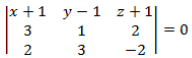0 = (x + 1) (- 2 – 6) – (y – 1) (- 6 – 4) + (z + 1) (9 – 2)

0 = (x + 1) (- 8) – (y – 1) (- 10) + (z + 1) (7)

0 = – 8x – 8 + 10y – 10 + 7z + 7

8x + 8 – 10y + 10 – 7z – 7 = 0

8x – 10y – 7z + 11 = 0

OR

[b] x11 − x6 + x5 − 1 = 0

x6 [x5 – 1] [x5 – 1] = 0

x = (-1) = (cis 𝛑)

= (cis (2k𝛑 + 𝛑)) k = 0, 1, 2, 3, 4, 5

x = cis 𝛑 / 6, cis 3𝛑 / 6, cis 5𝛑 / 6, cis 7𝛑 / 6, cis 9𝛑 / 6, cis 11𝛑 / 6

x = (cis 0)

= (cis (2k𝛑))

= cis 2k𝛑 / 5 , k = 0, 1, 2, 3, 4, 5

x = 0, cis 2𝛑 / 5, cis 4𝛑 / 5, cis 6𝛑 / 5, cis 8𝛑 / 5.

Question 43: [a] Show that the sum of the focal distances of any point on an ellipse is equal to the length of the major axis and also prove that the locus of a point which moves so that the sum of its distances from (3, 0) and (−3, 0) is 9, is x2 / (81 / 4) + y2 / (45 / 4) = 1.

OR

(b) Prove that the area of the largest rectangle that can be inscribed in a circle of radius ‘r’ is 2r2.

Solution:

[a]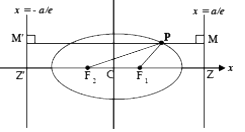F1P + F2P = 2a

x = a / e, x = – a / e

F1P / PM = e

F2P / PM = e

F1P = e PM

F2P = e PM

F1P + F2P = e (PM + PM)

= e (MM’)

= e (2a / e)

= 2a

= length of major axis

2a = 9

a = 9 / 2

ae = 3

b2 = a2 – (ae)2

= (81 / 4) – 9

= 45 / 4

The equation is x2 / (81 / 4) + y2 / (45 / 4) = 1.

OR

[b] x = 2r cos θ

y = 2r sin θ

Area of rectangle = 2x.2y

A (θ) = 2r cosθ . 2r sinθ

d (A(θ)) / dθ = 4𝑟2 cos 2θ

d (A(θ)) / dθ = 0 then θ = 𝜋 / 4

A ‘’ (θ) = – 8r2 sin 2θ < 0 for θ = 𝜋 / 4

So A is maximum for θ = 𝜋 / 4

X = y = √2r,

Required area = x.y

= 2r. 2r

= 2r2

Question 44: [a] A missile fired from ground level rises x metres vertically upwards in t seconds and x = 100t – (25 / 2)t2.

Find :

(i) the initial velocity of the missile

(ii) the time when the height of the missile is a maximum

(iii) the maximum height reached

(iv) the velocity with which the missile strikes the ground

OR

(b) Find the centre, foci and vertices of the hyperbola 16x2 − 9y2 − 32x − 18y + 151 = 0 and draw the diagram.

Solution:

[a] x = 100t – (25 / 2)t2

(i) To find the initial velocity of the missile.

So, t = 0

v = dx / dt = 100 – (25 / 2) 2t

v = 100 – 0

=100

(ii) The time when the height of the missile is a maximum is given by v = 0.

100 – 25t = 0

t = 4 seconds

(iii) The maximum height reached when t = 4 seconds

x = 100 (4) – (25 / 2)16x

= 200 meters

(iv) velocity if t = 4 + 4 = 8 seconds

v = 100 – 25(8)

= -100 m/sec

[b] equation (y + 1)2 / 16 − (x − 1)2 / 9 = 1

a2 = 16

b2 = 9

e = 5 / 4

Centre: (1, – 1)

Foci: (1, 4), (1, – 6)

Vertices : (1, 3), (1, – 4)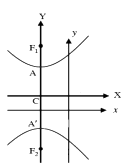Question 45: [a] The mean score of 1000 students for an examination is 34 and the standard deviation is 16. Determine the limit of the marks of the central 70% of the candidates by assuming the distribution is normal. P [0 < Z < 1.04] = 0.35

OR

(b) Compute the area between the curve y = sinx and y = cosx and the lines x = 0 and x = π.

Solution:

[a] a) P (Z1 < Z < Z2) = 70%

P (Z1 < Z < Z2) = 0.70

P(Z1 < Z < 0) = 0.35

P (0 < Z < Z2) = 0.35

Z1 and Z2 lie on the left and right side of the normal curve.

Z1 = −1.04 and Z2 = 1.04

Z = [x − 𝜇] / σ

= [x − 34] / 16

= – 1.04

X = 17.36

Z = x − 34 / 16 = 1.04

x = 50.64

70% of the students’ score lies between 17.36 and 50.64.

[b] y = sinx

y = cosx

Then sinx = cosx

→ x = 𝛑 / 4 ∈ (0, 𝛑)

Required area = ∫0𝛑/4 (cosx − sinx) dx + ∫𝛑/4𝛑 (sinx − cosx) dx

= 2 √2 square units

Question 46: [a] If w = x + 2y + z2 and x = cos t; y = sint; z = t find dw / dt by using chain rule. Also find dw / dt by substitution of x, y and z in w and hence verify the result.

OR

(b) A cup of tea at temperature 100oC is placed in a room whose temperature is 15oC and it cools to 60oC in 5 minutes. Find its temperature after a further interval of 5 minutes.

Solution:

[a] 𝑑𝑤 / 𝑑𝑡 = (𝜕𝑤 / 𝜕𝑥) (𝑑𝑥 / 𝑑𝑡) + (𝜕𝑤 / 𝜕𝑦) (𝑑𝑦 / 𝑑𝑡) + (𝜕𝑤 / 𝜕𝑧) (𝑑𝑧 / 𝑑𝑡)

= – sint + 2 cost + 2t …. (1)

w = cost + 2 sint + t2

𝑑𝑤 / 𝑑𝑡 = – sint + 2 cost + 2t …. (2)

b) dT / dt = k (T – s) , s = 15

T = s + c𝑒 𝑘𝑡……. (1)

t = 0 then T = 100

(1) => c = 85

t = 5, T = 60 then e5𝑘 = 45 / 85

t =10 , then T= ?

T = 15 + 83 e10𝑘 = 38.82

Question 47: [a] State all the five properties of groups.

OR

(b) Prove that the solution of the differential equation : (5D2 − 8D − 4)y = 5e(-⅖x) + 2ex + 3 is y = Ae2x + Be(-⅖x) – (5 / 12) xe(-⅖x) – (2 / 7) ex – (3 / 4).

Solution:

[a]

(i) Identity element of group is unique

(ii) Inverse of each element of group is unique

(iii) Reversal law

(iv) Cancellation law

(v) [a-1] − 1 = a

b) C.F = A𝑒2𝑥 + B𝑒 (−2/5)𝑥

P.I1 = (- 5 / 12) x𝑒 (−2/5)𝑥

P.I2 = (- 2 / 7) 𝑒𝑥

P.I3 = – 3 / 4

General solution y = C.F + P.I1 + P.I2 + P.I3

= A𝑒2𝑥 + B𝑒 (−2/5)𝑥 + (- 5 / 12) x𝑒 (−2/5)𝑥 + (- 2 / 7) 𝑒𝑥 (- 3 / 4)